Скачать презентацию Costs Cost is not a simple concept It

• Количество слайдов: 78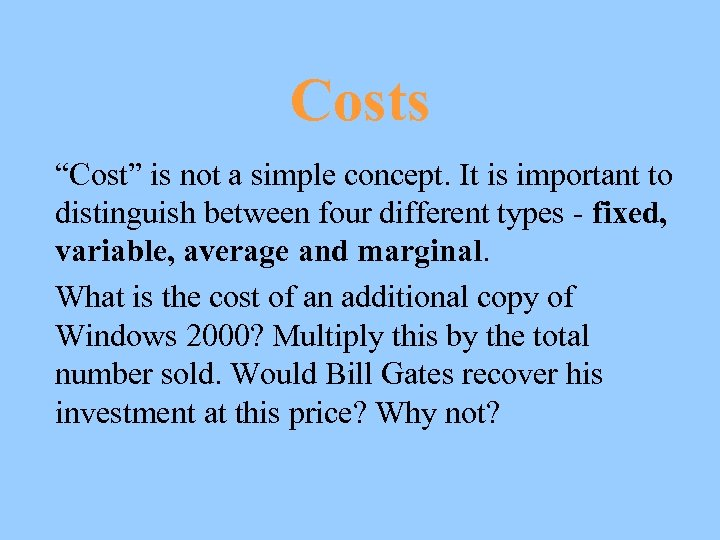Costs “Cost” is not a simple concept. It is important to distinguish between four different types - fixed, variable, average and marginal. What is the cost of an additional copy of Windows 2000? Multiply this by the total number sold. Would Bill Gates recover his investment at this price? Why not?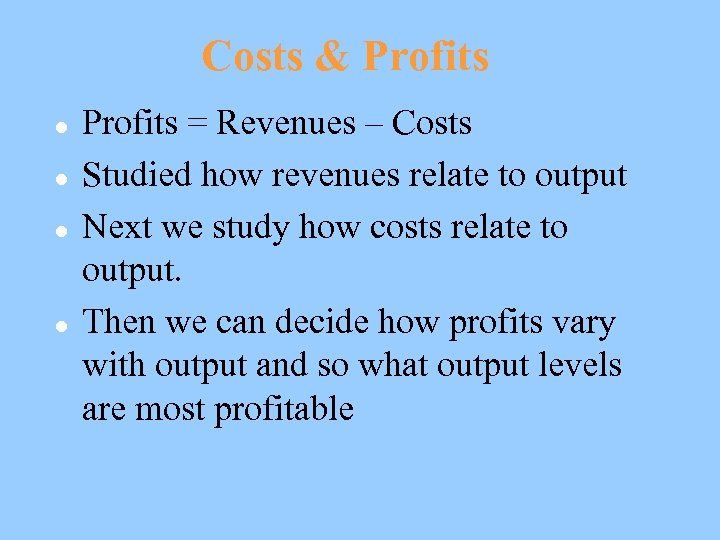Costs & Profits l l Profits = Revenues – Costs Studied how revenues relate to output Next we study how costs relate to output. Then we can decide how profits vary with output and so what output levels are most profitable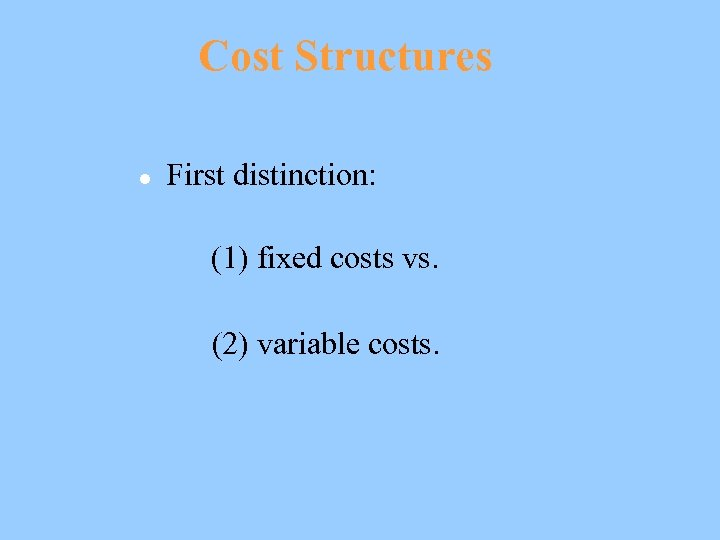Cost Structures l First distinction: (1) fixed costs vs. (2) variable costs.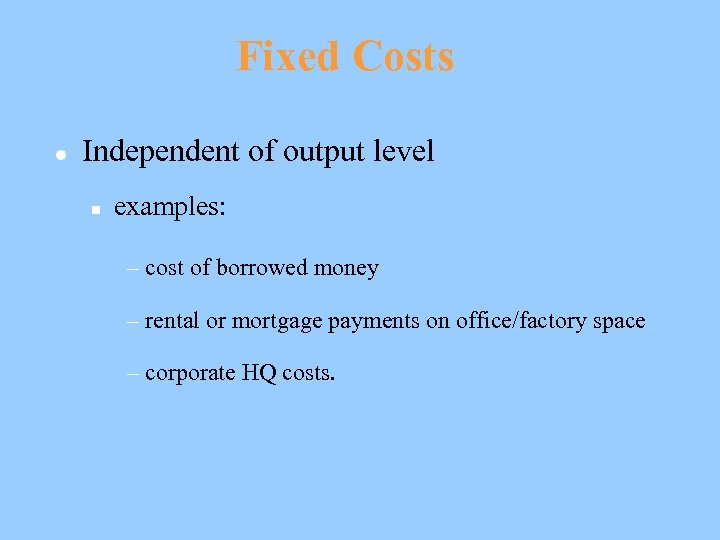Fixed Costs l Independent of output level n examples: – cost of borrowed money – rental or mortgage payments on office/factory space – corporate HQ costs.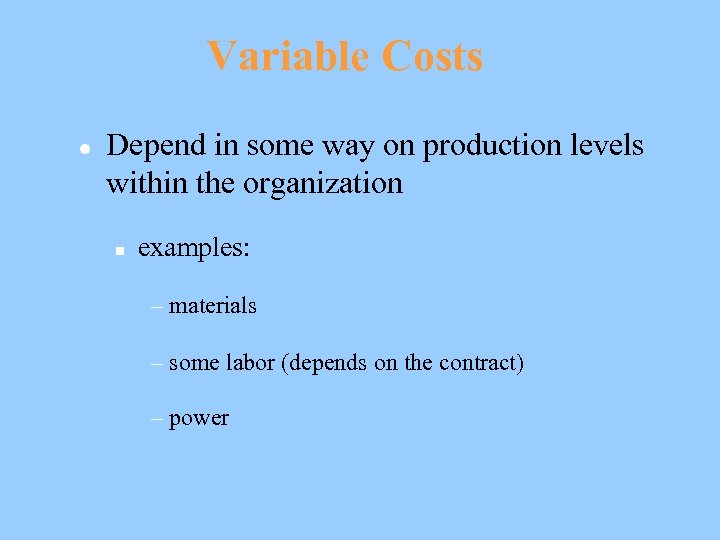Variable Costs l Depend in some way on production levels within the organization n examples: – materials – some labor (depends on the contract) – power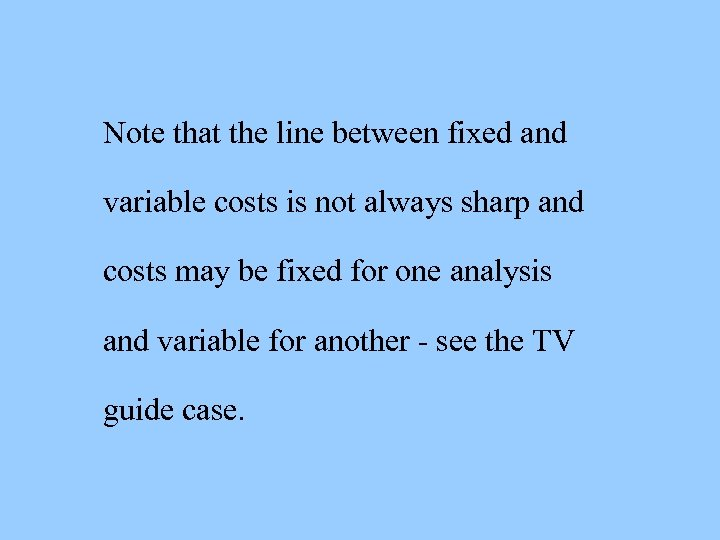Note that the line between fixed and variable costs is not always sharp and costs may be fixed for one analysis and variable for another - see the TV guide case.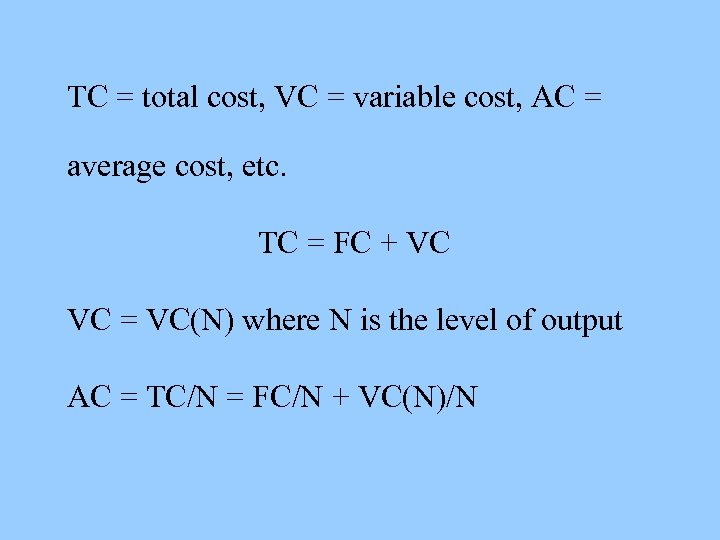TC = total cost, VC = variable cost, AC = average cost, etc. TC = FC + VC VC = VC(N) where N is the level of output AC = TC/N = FC/N + VC(N)/N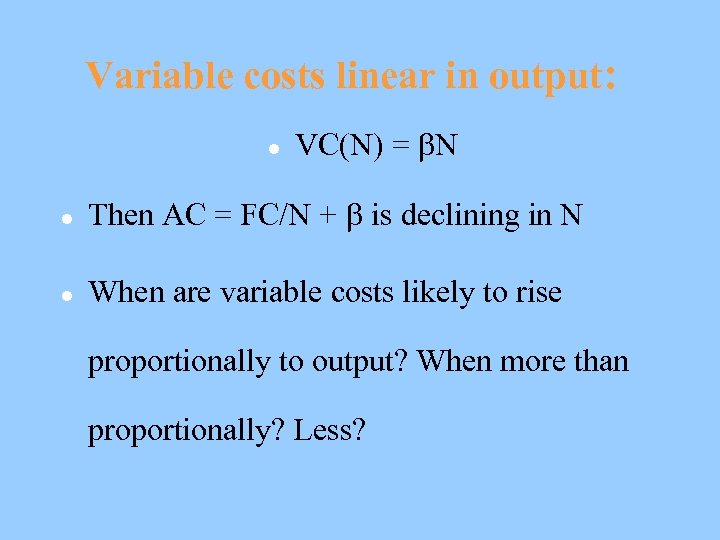Variable costs linear in output: l VC(N) = N l Then AC = FC/N + is declining in N l When are variable costs likely to rise proportionally to output? When more than proportionally? Less?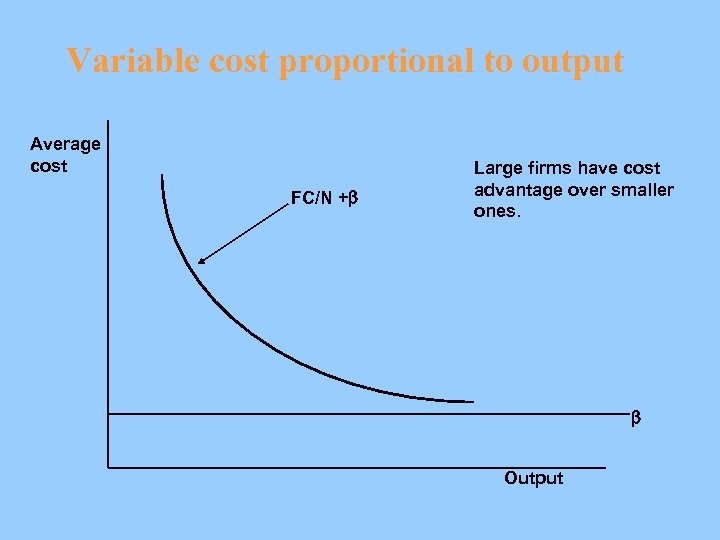Variable cost proportional to output Average cost FC/N +b Large firms have cost advantage over smaller ones. b Output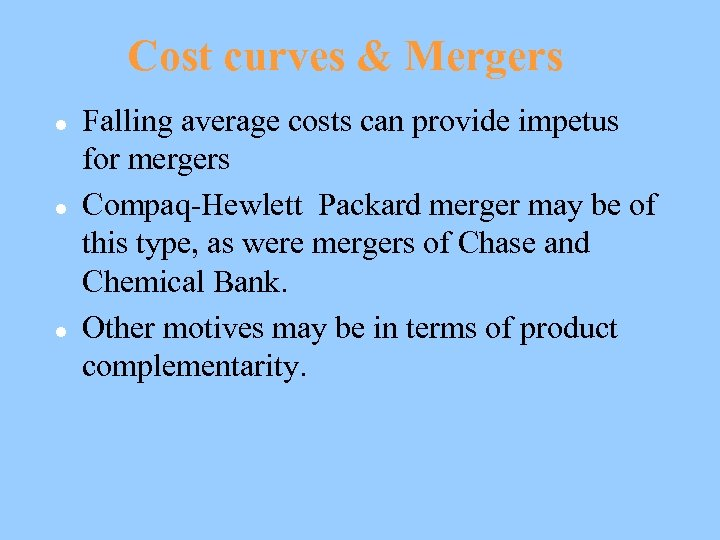Cost curves & Mergers l l l Falling average costs can provide impetus for mergers Compaq-Hewlett Packard merger may be of this type, as were mergers of Chase and Chemical Bank. Other motives may be in terms of product complementarity.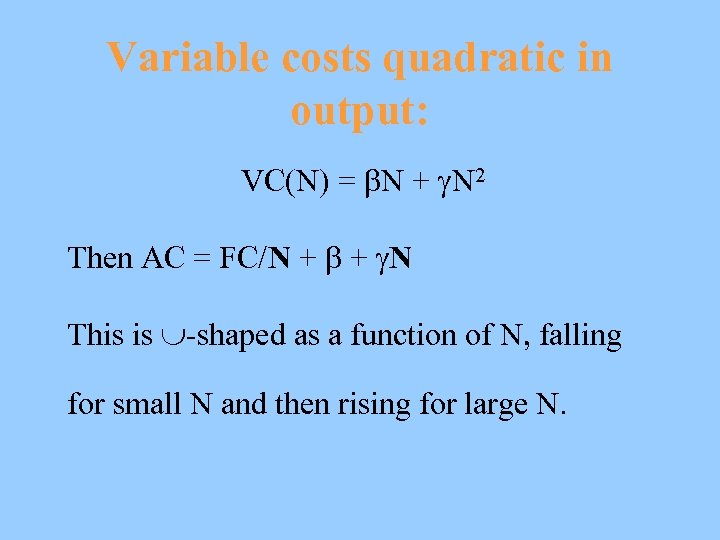Variable costs quadratic in output: VC(N) = N + N 2 Then AC = FC/N + + N This is -shaped as a function of N, falling for small N and then rising for large N.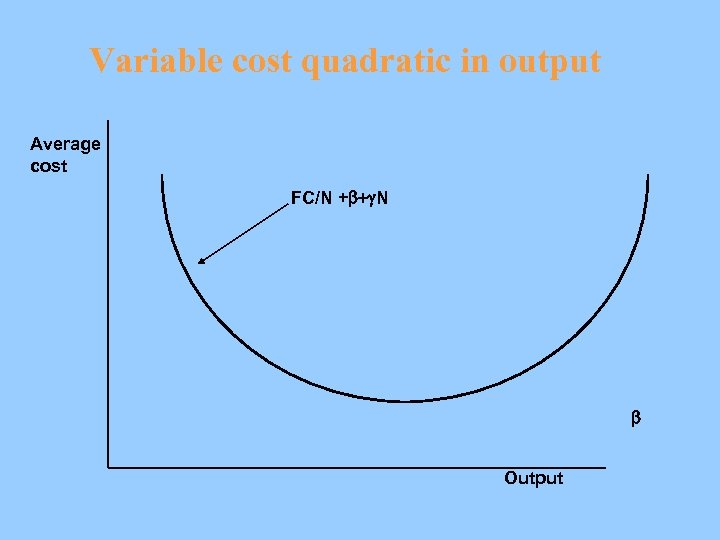Variable cost quadratic in output Average cost FC/N +b+g. N b Output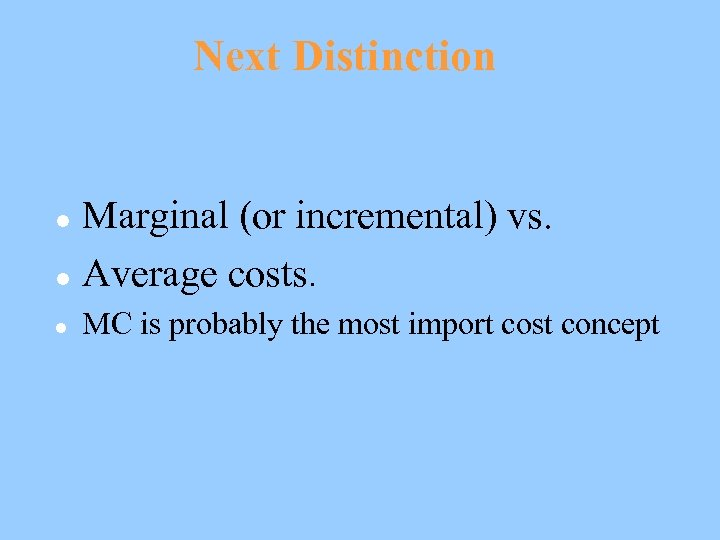Next Distinction Marginal (or incremental) vs. l Average costs. l l MC is probably the most import cost concept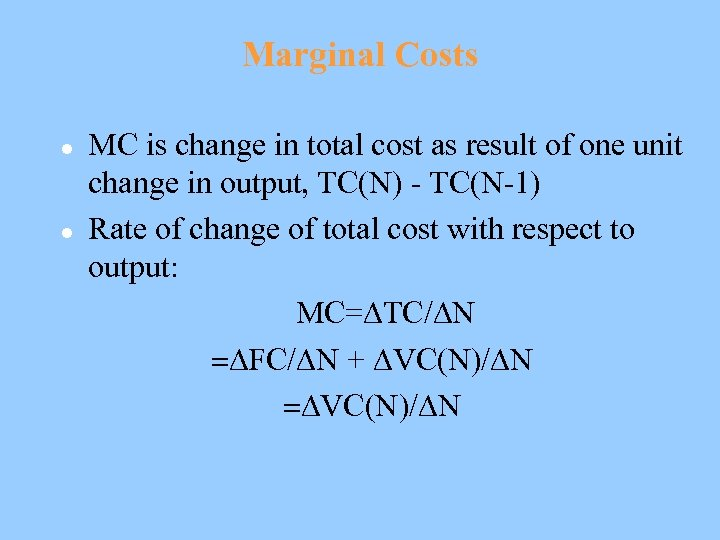Marginal Costs l l MC is change in total cost as result of one unit change in output, TC(N) - TC(N-1) Rate of change of total cost with respect to output: MC= TC/ N = FC/ N + VC(N)/ N = VC(N)/ N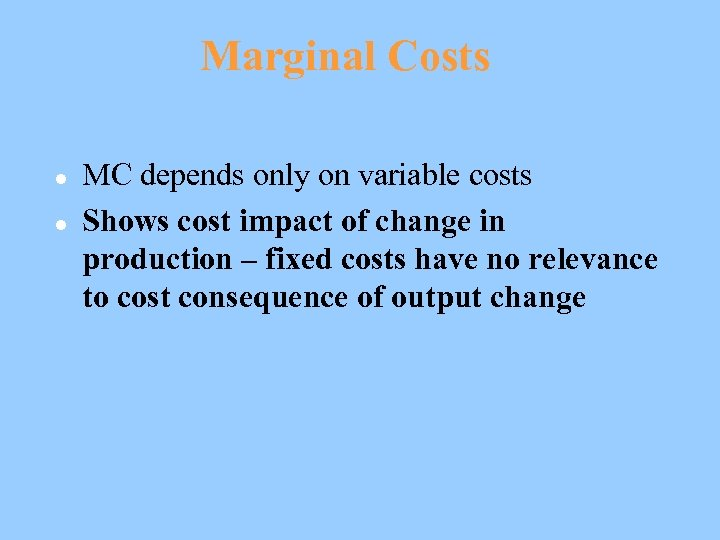Marginal Costs l l MC depends only on variable costs Shows cost impact of change in production – fixed costs have no relevance to cost consequence of output change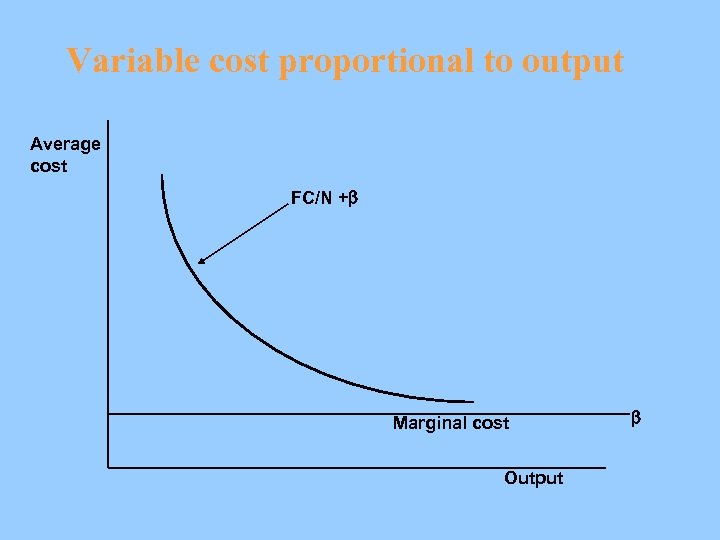Variable cost proportional to output Average cost FC/N +b Marginal cost Output b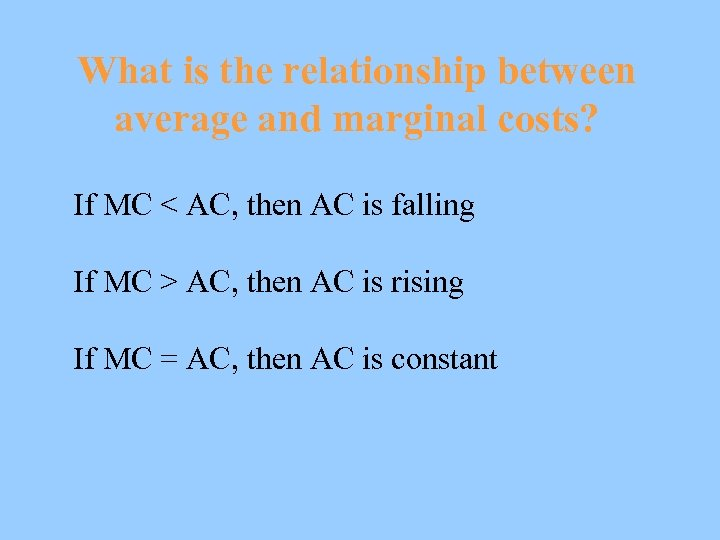What is the relationship between average and marginal costs? If MC < AC, then AC is falling If MC > AC, then AC is rising If MC = AC, then AC is constant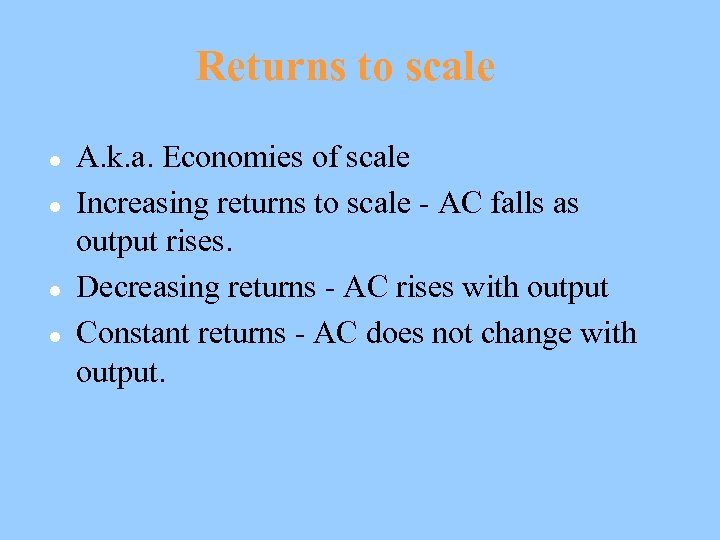Returns to scale l l A. k. a. Economies of scale Increasing returns to scale - AC falls as output rises. Decreasing returns - AC rises with output Constant returns - AC does not change with output.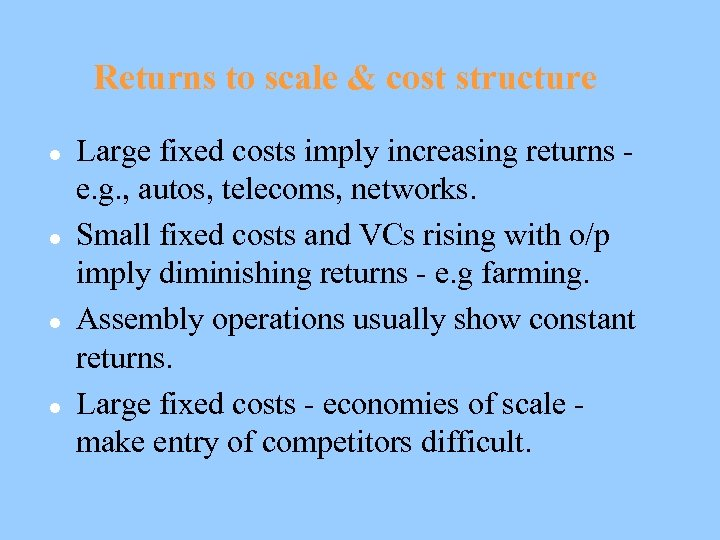Returns to scale & cost structure l l Large fixed costs imply increasing returns e. g. , autos, telecoms, networks. Small fixed costs and VCs rising with o/p imply diminishing returns - e. g farming. Assembly operations usually show constant returns. Large fixed costs - economies of scale make entry of competitors difficult.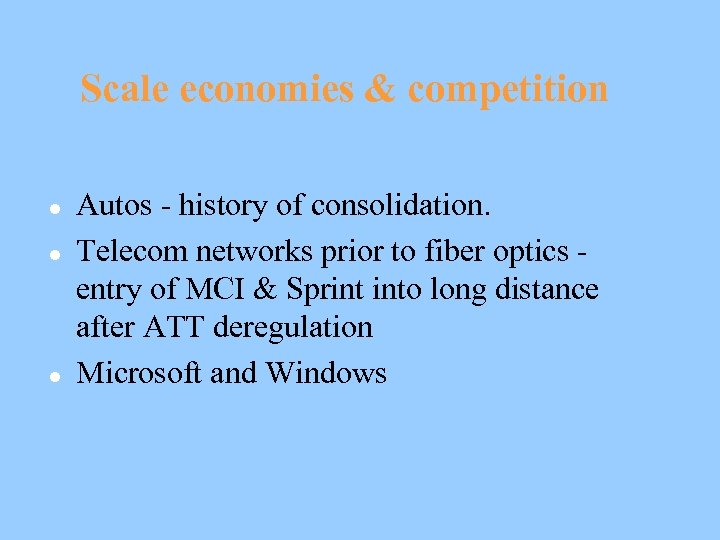Scale economies & competition l l l Autos - history of consolidation. Telecom networks prior to fiber optics entry of MCI & Sprint into long distance after ATT deregulation Microsoft and Windows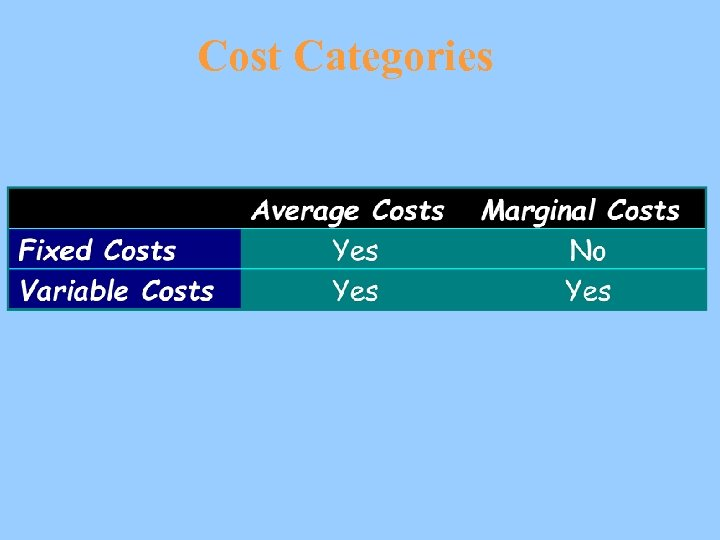Cost Categories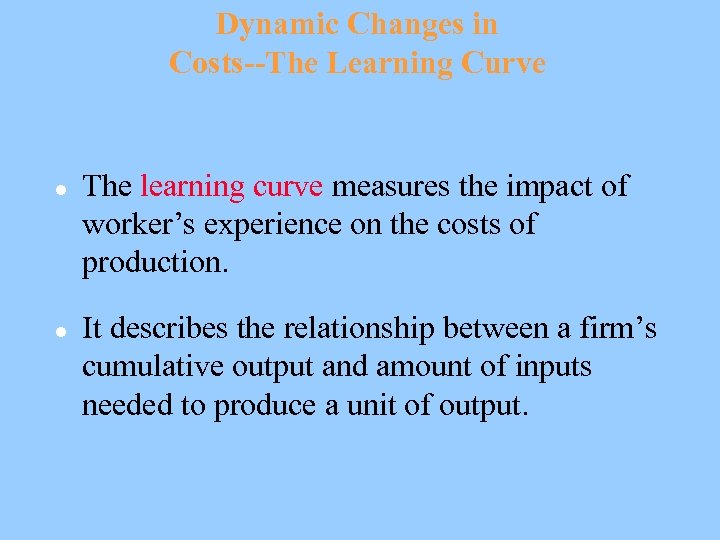Dynamic Changes in Costs--The Learning Curve l l The learning curve measures the impact of worker’s experience on the costs of production. It describes the relationship between a firm’s cumulative output and amount of inputs needed to produce a unit of output.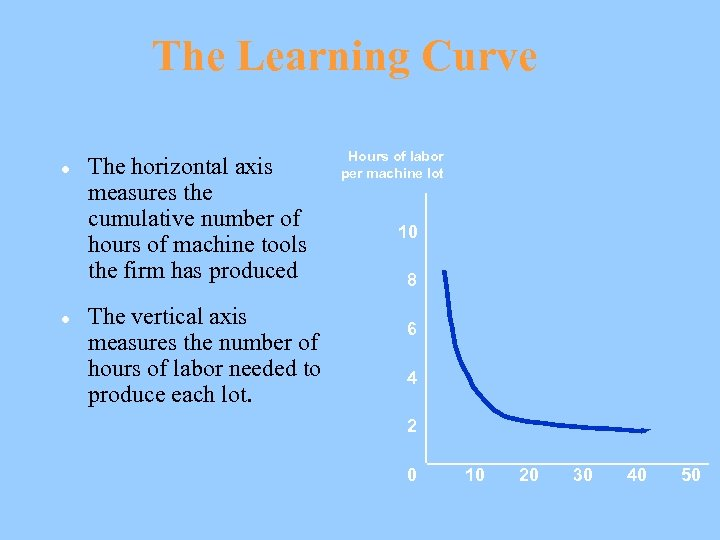The Learning Curve l l The horizontal axis measures the cumulative number of hours of machine tools the firm has produced The vertical axis measures the number of hours of labor needed to produce each lot. Hours of labor per machine lot 10 8 6 4 2 0 10 20 30 40 50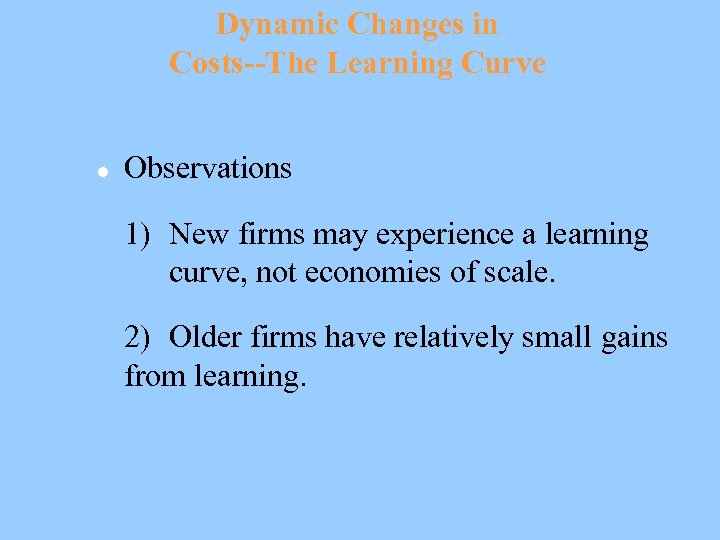Dynamic Changes in Costs--The Learning Curve l Observations 1) New firms may experience a learning curve, not economies of scale. 2) Older firms have relatively small gains from learning.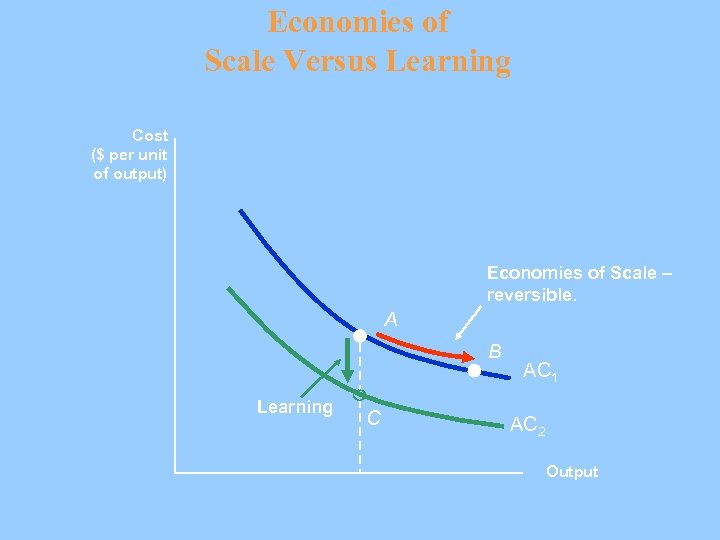Economies of Scale Versus Learning Cost (\$ per unit of output) Economies of Scale – reversible. A B Learning C AC 1 AC 2 Output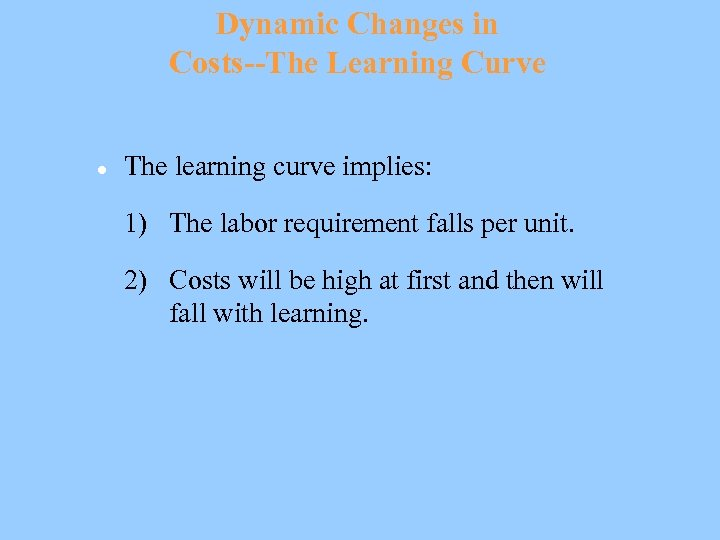Dynamic Changes in Costs--The Learning Curve l The learning curve implies: 1) The labor requirement falls per unit. 2) Costs will be high at first and then will fall with learning.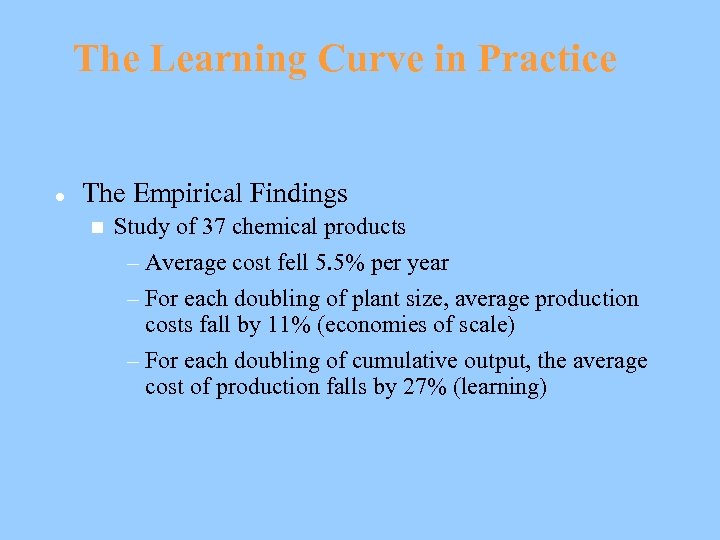The Learning Curve in Practice l The Empirical Findings n Study of 37 chemical products – Average cost fell 5. 5% per year – For each doubling of plant size, average production costs fall by 11% (economies of scale) – For each doubling of cumulative output, the average cost of production falls by 27% (learning)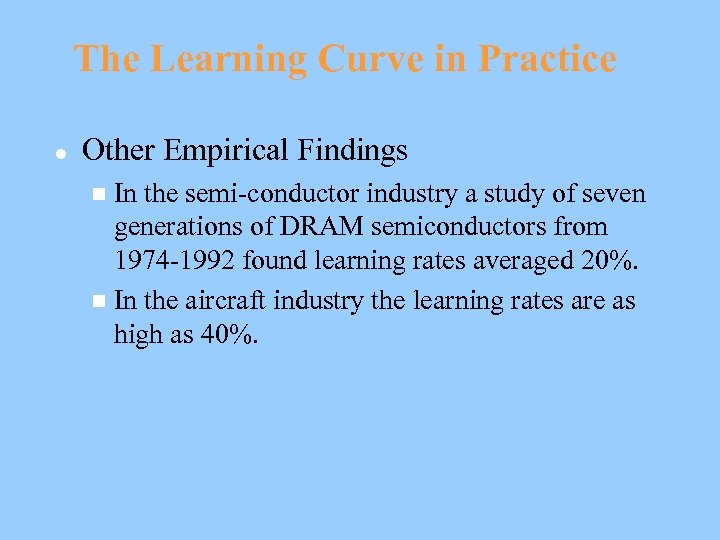The Learning Curve in Practice l Other Empirical Findings In the semi-conductor industry a study of seven generations of DRAM semiconductors from 1974 -1992 found learning rates averaged 20%. n In the aircraft industry the learning rates are as high as 40%. n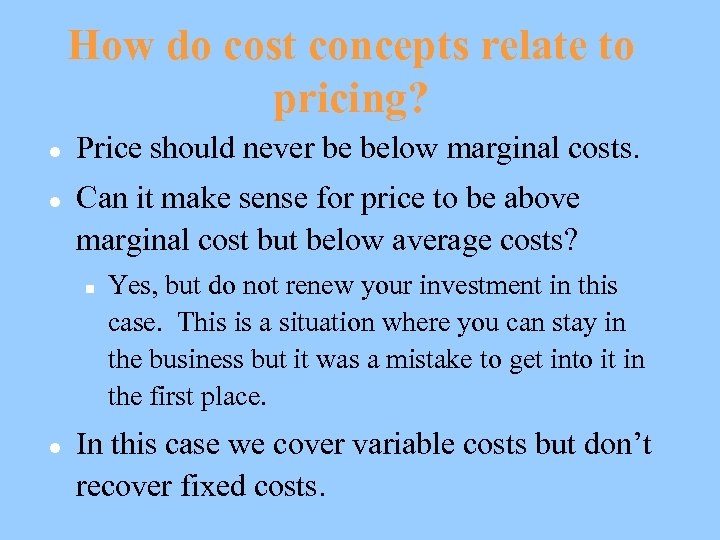How do cost concepts relate to pricing? l l Price should never be below marginal costs. Can it make sense for price to be above marginal cost but below average costs? n l Yes, but do not renew your investment in this case. This is a situation where you can stay in the business but it was a mistake to get into it in the first place. In this case we cover variable costs but don’t recover fixed costs.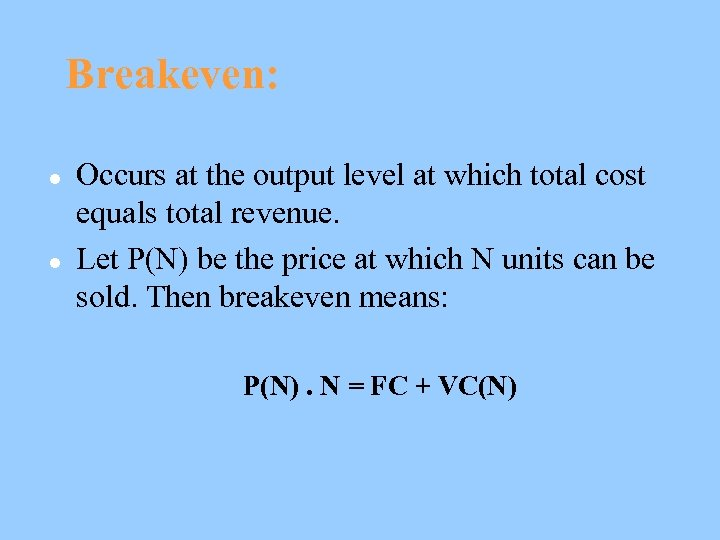Breakeven: l l Occurs at the output level at which total cost equals total revenue. Let P(N) be the price at which N units can be sold. Then breakeven means: P(N). N = FC + VC(N)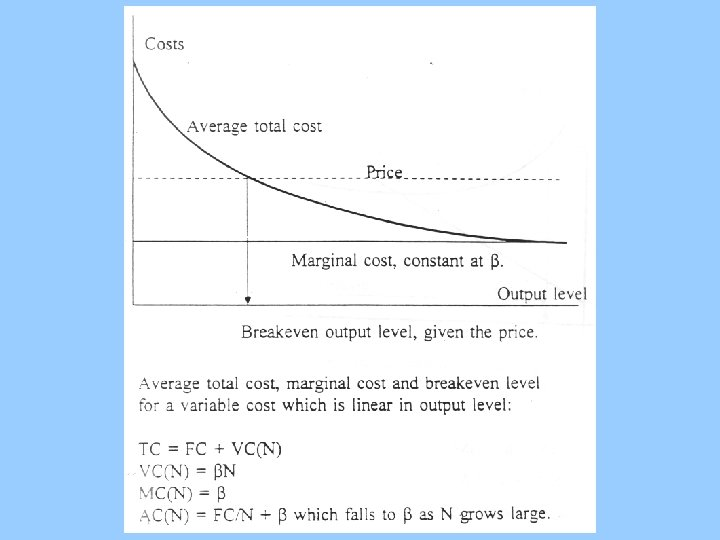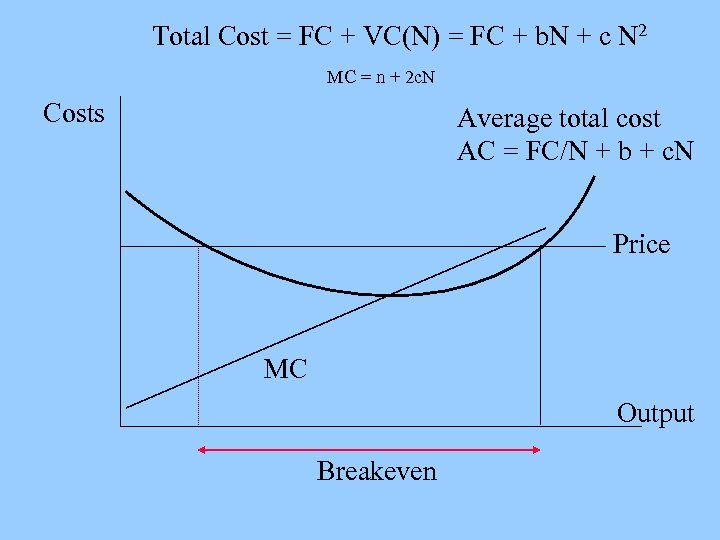Total Cost = FC + VC(N) = FC + b. N + c N 2 MC = n + 2 c. N Costs Average total cost AC = FC/N + b + c. N Price MC Output Breakeven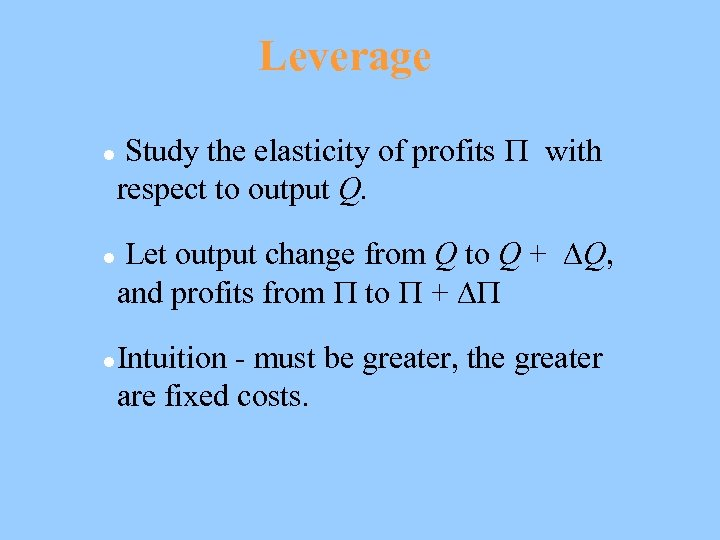Leverage l l l Study the elasticity of profits with respect to output Q. Let output change from Q to Q + Q, and profits from to + Intuition - must be greater, the greater are fixed costs.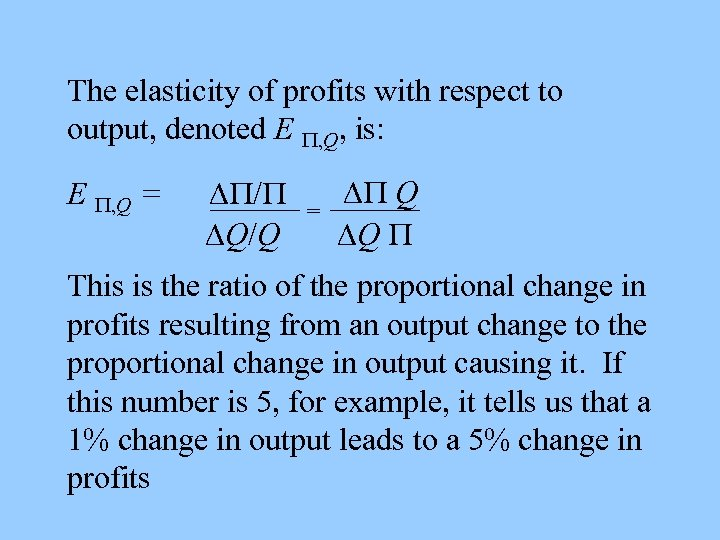The elasticity of profits with respect to output, denoted E , Q, is: E , Q = / Q/Q = Q Q This is the ratio of the proportional change in profits resulting from an output change to the proportional change in output causing it. If this number is 5, for example, it tells us that a 1% change in output leads to a 5% change in profits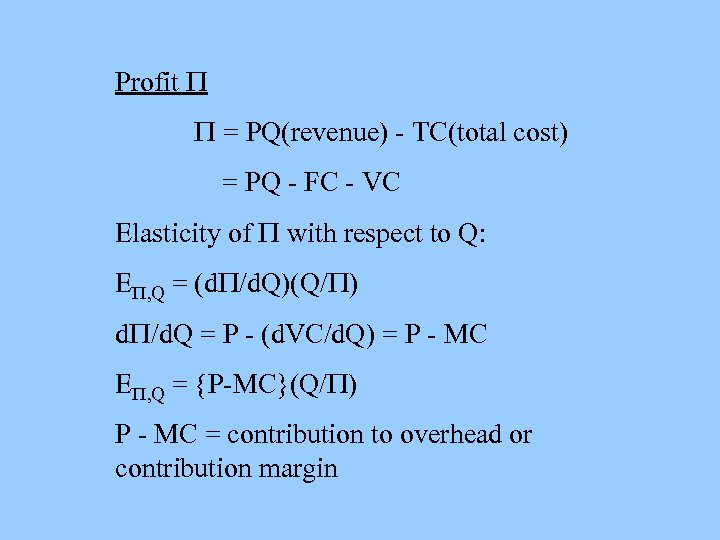Profit = PQ(revenue) - TC(total cost) = PQ - FC - VC Elasticity of with respect to Q: E , Q = (d /d. Q)(Q/ ) d /d. Q = P - (d. VC/d. Q) = P - MC E , Q = P-MC (Q/ ) P - MC = contribution to overhead or contribution margin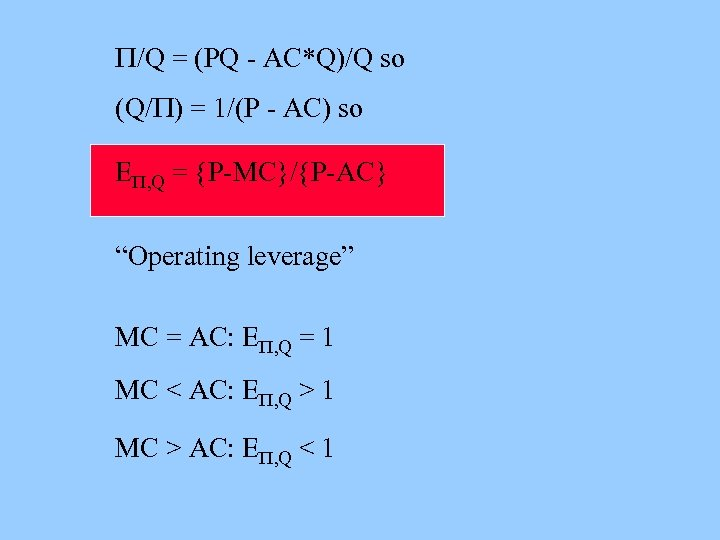/Q = (PQ - AC*Q)/Q so (Q/ ) = 1/(P - AC) so E , Q = P-MC / P-AC “Operating leverage” MC = AC: E , Q = 1 MC < AC: E , Q > 1 MC > AC: E , Q < 1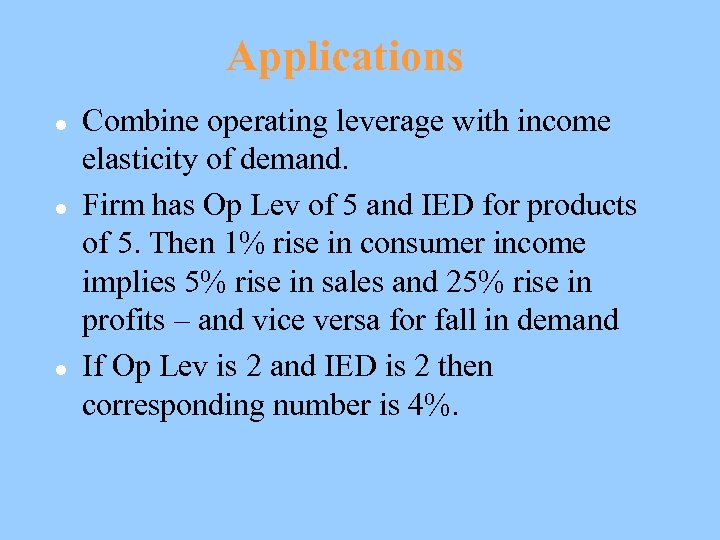Applications l l l Combine operating leverage with income elasticity of demand. Firm has Op Lev of 5 and IED for products of 5. Then 1% rise in consumer income implies 5% rise in sales and 25% rise in profits – and vice versa for fall in demand If Op Lev is 2 and IED is 2 then corresponding number is 4%.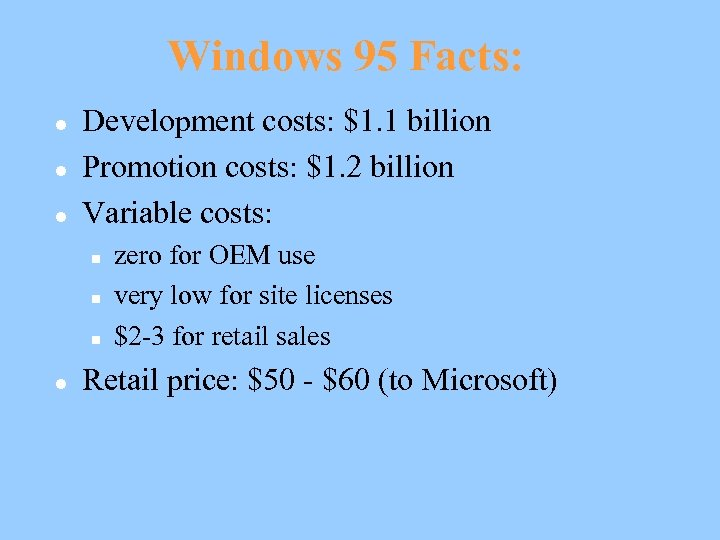Windows 95 Facts: l l l Development costs: \$1. 1 billion Promotion costs: \$1. 2 billion Variable costs: n n n l zero for OEM use very low for site licenses \$2 -3 for retail sales Retail price: \$50 - \$60 (to Microsoft)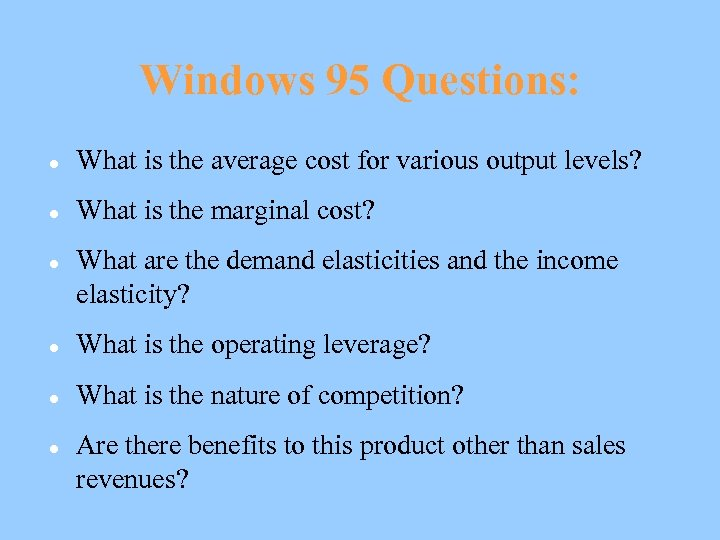Windows 95 Questions: l What is the average cost for various output levels? l What is the marginal cost? l What are the demand elasticities and the income elasticity? l What is the operating leverage? l What is the nature of competition? l Are there benefits to this product other than sales revenues?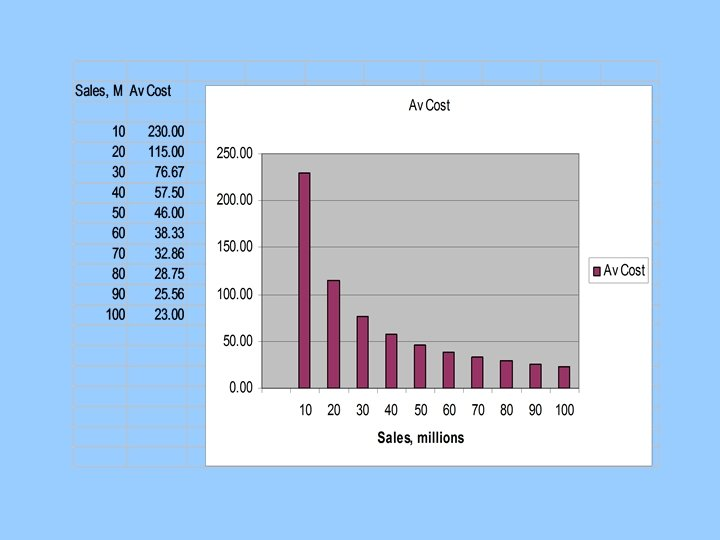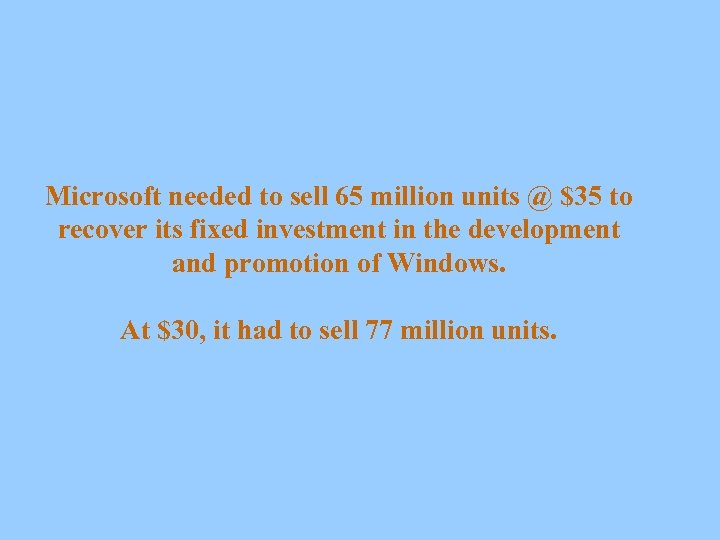Microsoft needed to sell 65 million units @ \$35 to recover its fixed investment in the development and promotion of Windows. At \$30, it had to sell 77 million units.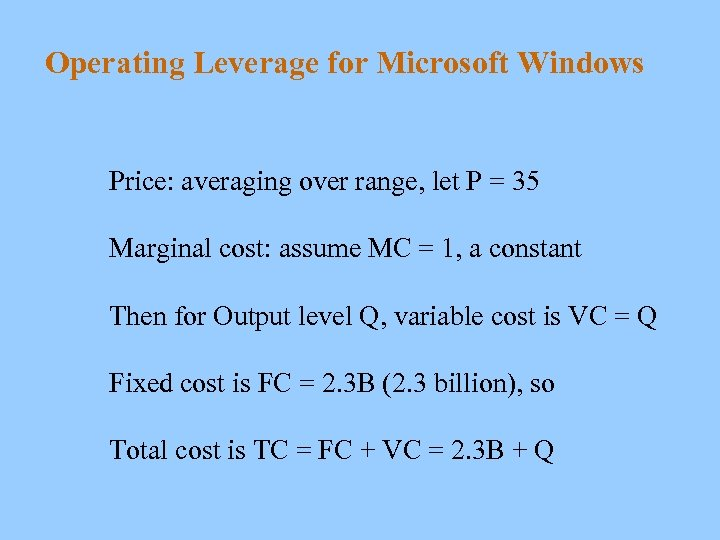Operating Leverage for Microsoft Windows Price: averaging over range, let P = 35 Marginal cost: assume MC = 1, a constant Then for Output level Q, variable cost is VC = Q Fixed cost is FC = 2. 3 B (2. 3 billion), so Total cost is TC = FC + VC = 2. 3 B + Q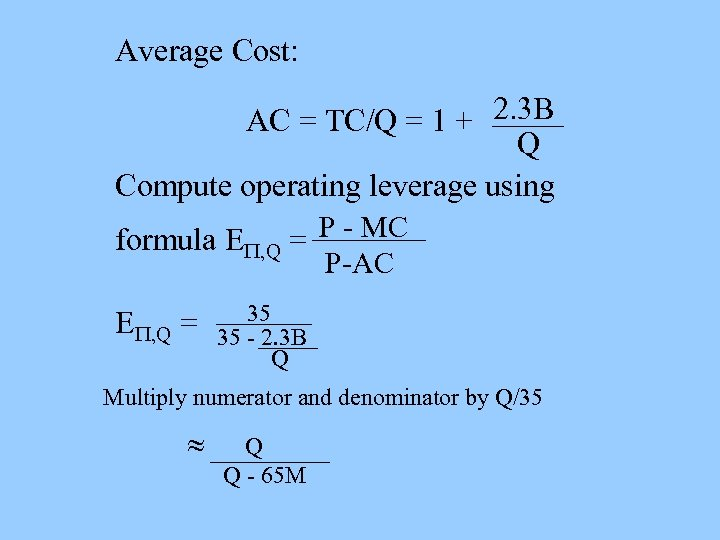Average Cost: AC = TC/Q = 1 + 2. 3 B Q Compute operating leverage using formula E , Q = P - MC P-AC E , Q = 35 35 - 2. 3 B Q Multiply numerator and denominator by Q/35 Q Q - 65 M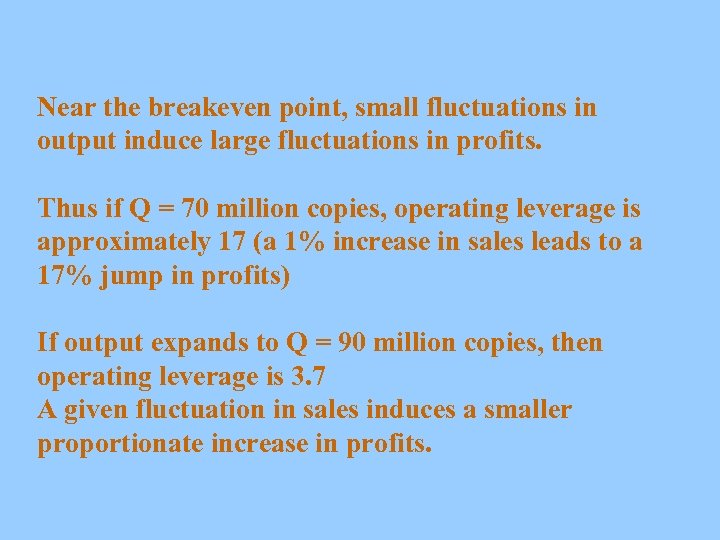Near the breakeven point, small fluctuations in output induce large fluctuations in profits. Thus if Q = 70 million copies, operating leverage is approximately 17 (a 1% increase in sales leads to a 17% jump in profits) If output expands to Q = 90 million copies, then operating leverage is 3. 7 A given fluctuation in sales induces a smaller proportionate increase in profits.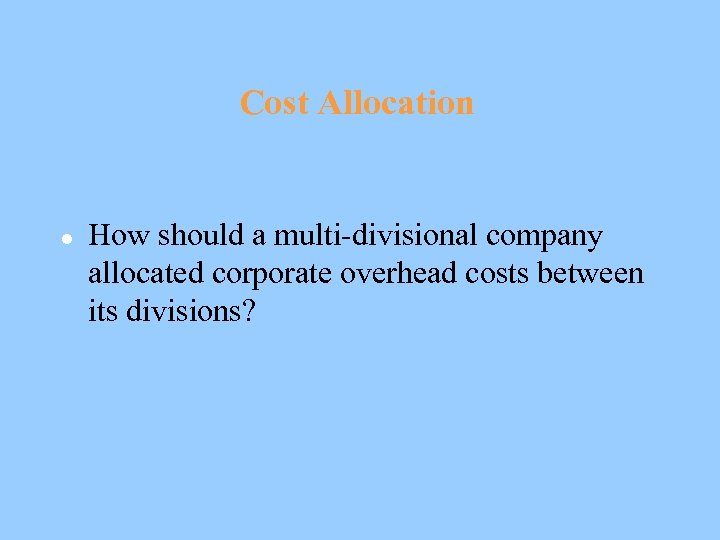Cost Allocation l How should a multi-divisional company allocated corporate overhead costs between its divisions?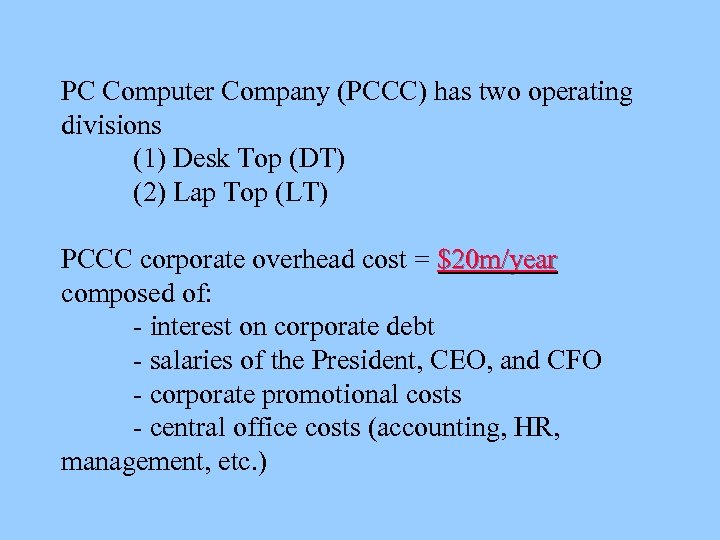PC Computer Company (PCCC) has two operating divisions (1) Desk Top (DT) (2) Lap Top (LT) PCCC corporate overhead cost = \$20 m/year composed of: - interest on corporate debt - salaries of the President, CEO, and CFO - corporate promotional costs - central office costs (accounting, HR, management, etc. )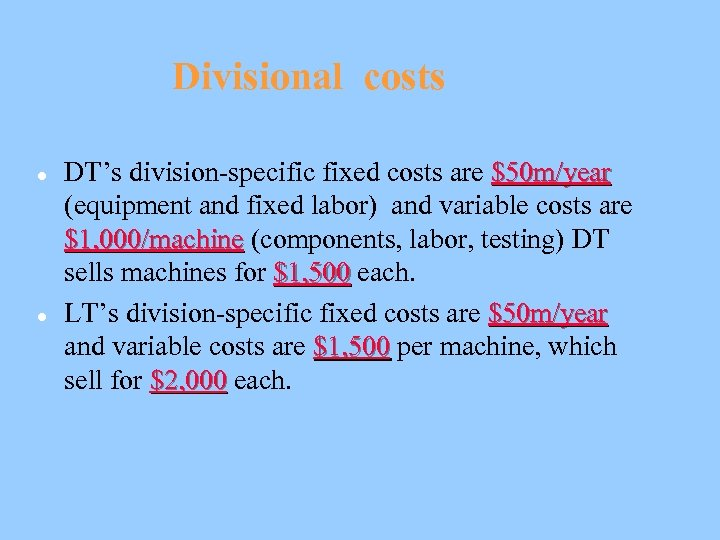Divisional costs l l DT’s division-specific fixed costs are \$50 m/year (equipment and fixed labor) and variable costs are \$1, 000/machine (components, labor, testing) DT sells machines for \$1, 500 each. LT’s division-specific fixed costs are \$50 m/year and variable costs are \$1, 500 per machine, which sell for \$2, 000 each.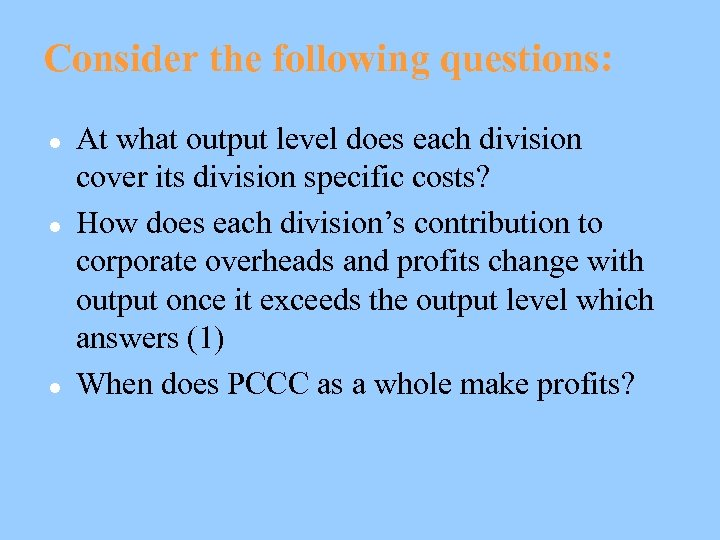Consider the following questions: l l l At what output level does each division cover its division specific costs? How does each division’s contribution to corporate overheads and profits change with output once it exceeds the output level which answers (1) When does PCCC as a whole make profits?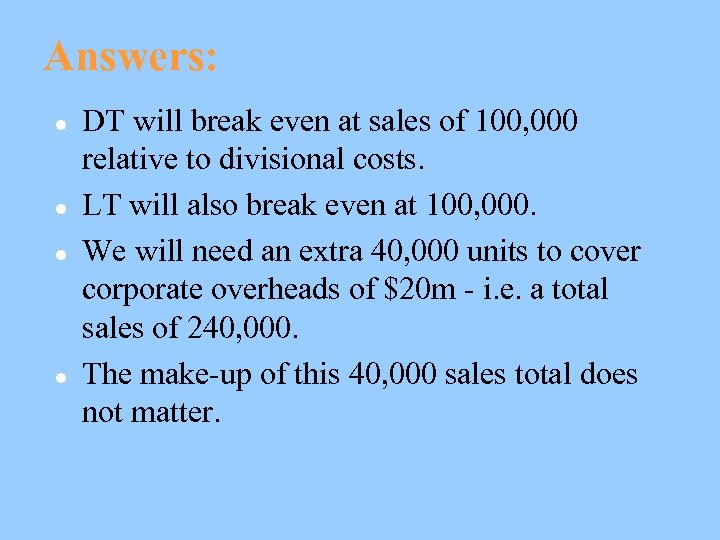Answers: l l DT will break even at sales of 100, 000 relative to divisional costs. LT will also break even at 100, 000. We will need an extra 40, 000 units to cover corporate overheads of \$20 m - i. e. a total sales of 240, 000. The make-up of this 40, 000 sales total does not matter.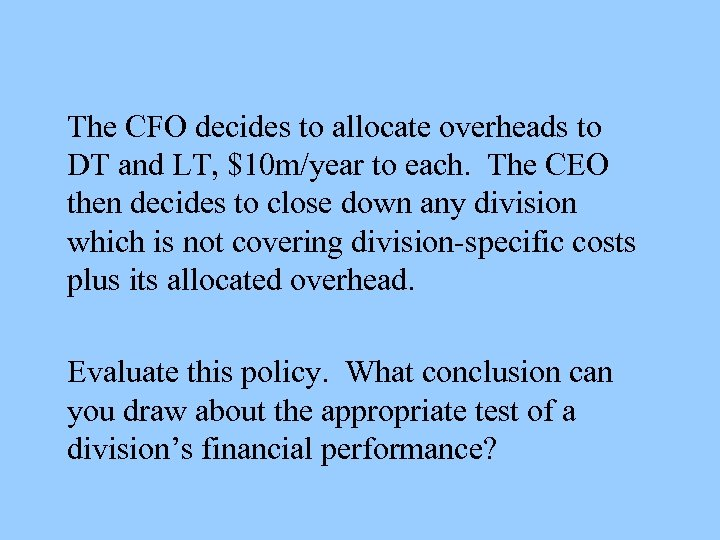The CFO decides to allocate overheads to DT and LT, \$10 m/year to each. The CEO then decides to close down any division which is not covering division-specific costs plus its allocated overhead. Evaluate this policy. What conclusion can you draw about the appropriate test of a division’s financial performance?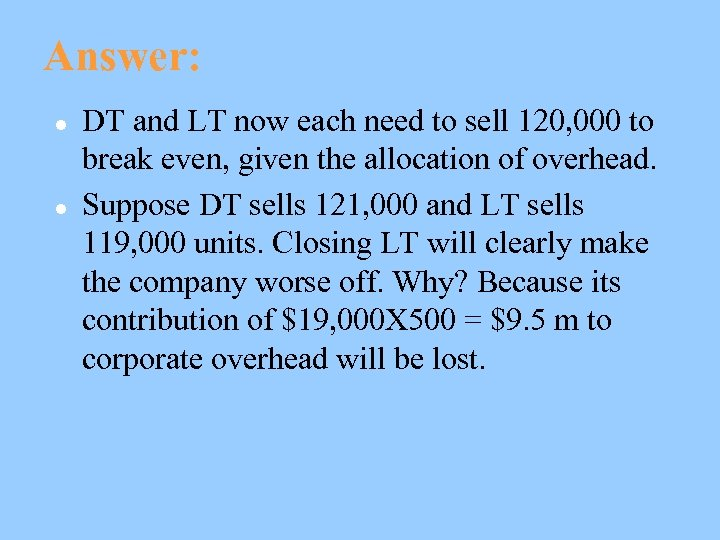Answer: l l DT and LT now each need to sell 120, 000 to break even, given the allocation of overhead. Suppose DT sells 121, 000 and LT sells 119, 000 units. Closing LT will clearly make the company worse off. Why? Because its contribution of \$19, 000 X 500 = \$9. 5 m to corporate overhead will be lost.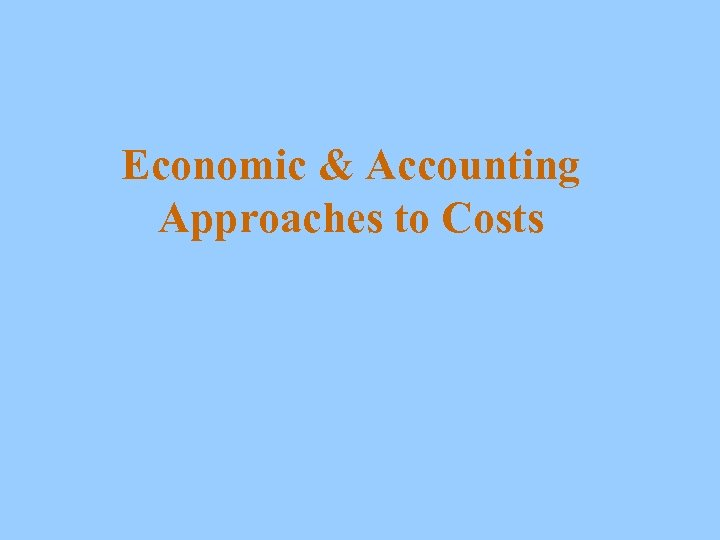Economic & Accounting Approaches to Costs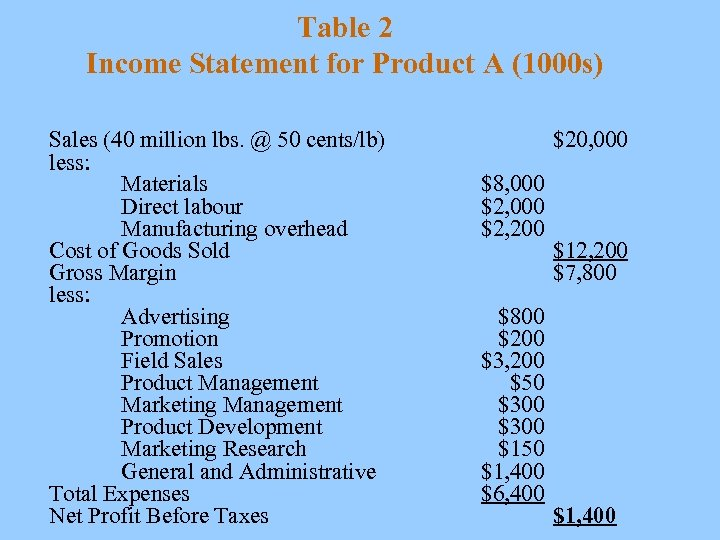Table 2 Income Statement for Product A (1000 s) Sales (40 million lbs. @ 50 cents/lb) less: Materials Direct labour Manufacturing overhead Cost of Goods Sold Gross Margin less: Advertising Promotion Field Sales Product Management Marketing Management Product Development Marketing Research General and Administrative Total Expenses Net Profit Before Taxes \$20, 000 \$8, 000 \$2, 200 \$800 \$200 \$3, 200 \$50 \$300 \$150 \$1, 400 \$6, 400 \$12, 200 \$7, 800 \$1, 400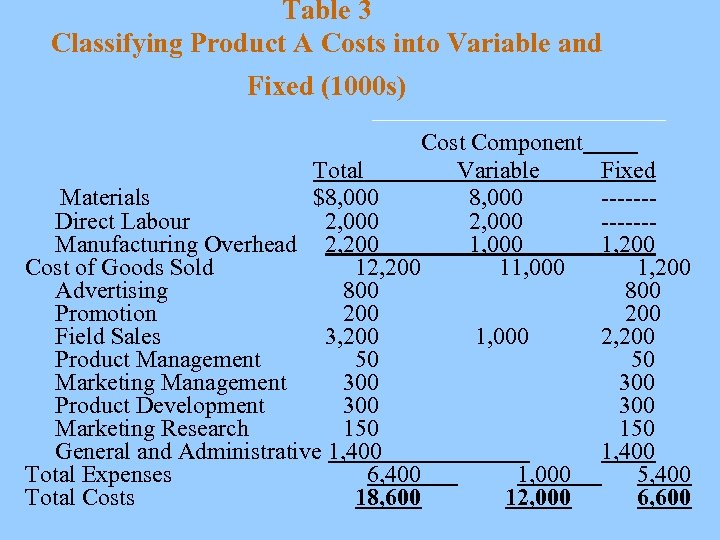Table 3 Classifying Product A Costs into Variable and Fixed (1000 s) Cost Component Total Variable Materials \$8, 000 Direct Labour 2, 000 Manufacturing Overhead 2, 200 1, 000 Cost of Goods Sold 12, 200 11, 000 Advertising 800 Promotion 200 Field Sales 3, 200 1, 000 Product Management 50 Marketing Management 300 Product Development 300 Marketing Research 150 General and Administrative 1, 400 Total Expenses 6, 400 1, 000 Total Costs 18, 600 12, 000 Fixed ------1, 200 800 2, 200 50 300 150 1, 400 5, 400 6, 600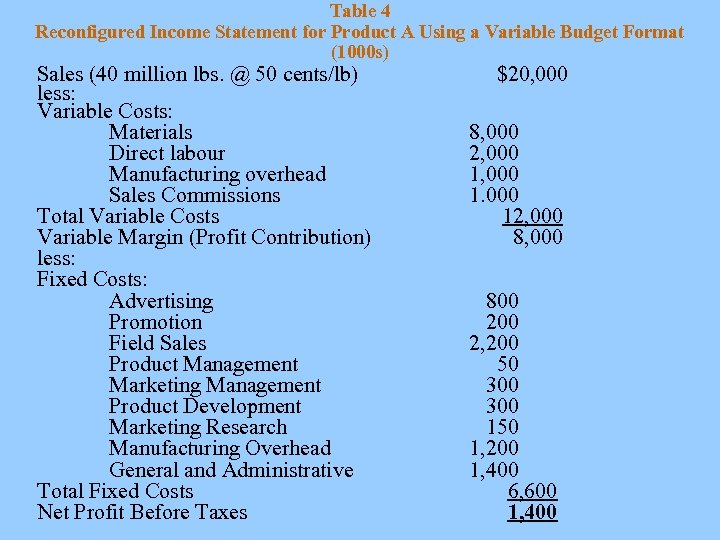Table 4 Reconfigured Income Statement for Product A Using a Variable Budget Format (1000 s) Sales (40 million lbs. @ 50 cents/lb) less: Variable Costs: Materials Direct labour Manufacturing overhead Sales Commissions Total Variable Costs Variable Margin (Profit Contribution) less: Fixed Costs: Advertising Promotion Field Sales Product Management Marketing Management Product Development Marketing Research Manufacturing Overhead General and Administrative Total Fixed Costs Net Profit Before Taxes \$20, 000 8, 000 2, 000 12, 000 800 2, 200 50 300 150 1, 200 1, 400 6, 600 1, 400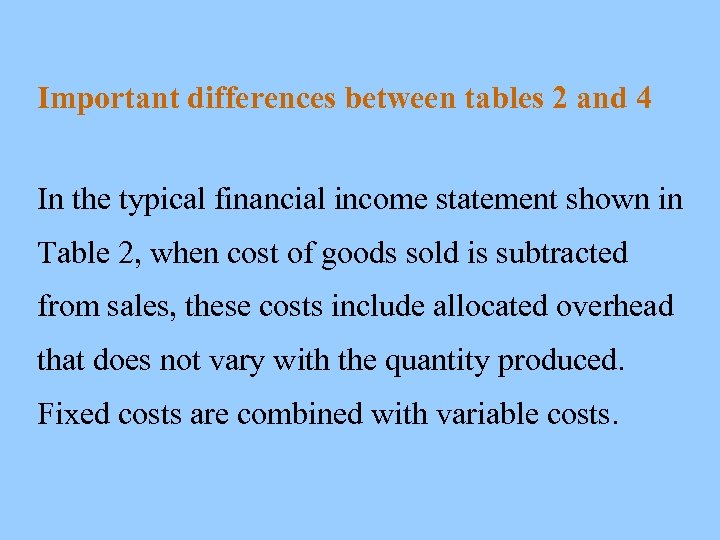Important differences between tables 2 and 4 In the typical financial income statement shown in Table 2, when cost of goods sold is subtracted from sales, these costs include allocated overhead that does not vary with the quantity produced. Fixed costs are combined with variable costs.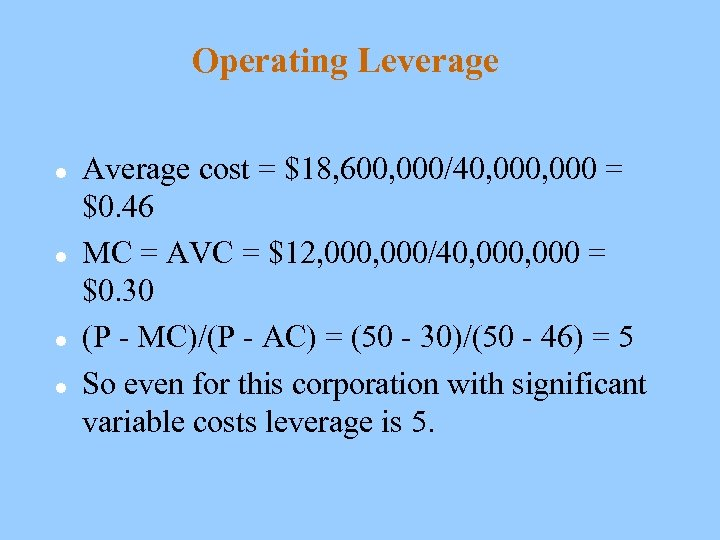Operating Leverage l l Average cost = \$18, 600, 000/40, 000 = \$0. 46 MC = AVC = \$12, 000/40, 000 = \$0. 30 (P - MC)/(P - AC) = (50 - 30)/(50 - 46) = 5 So even for this corporation with significant variable costs leverage is 5.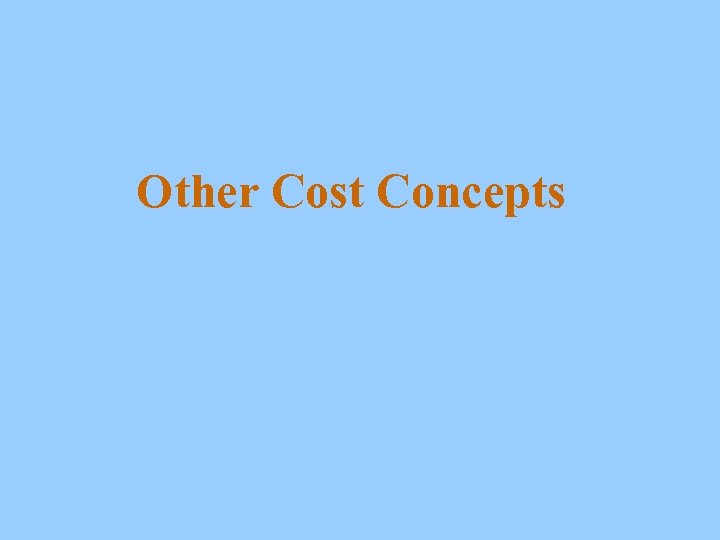Other Cost Concepts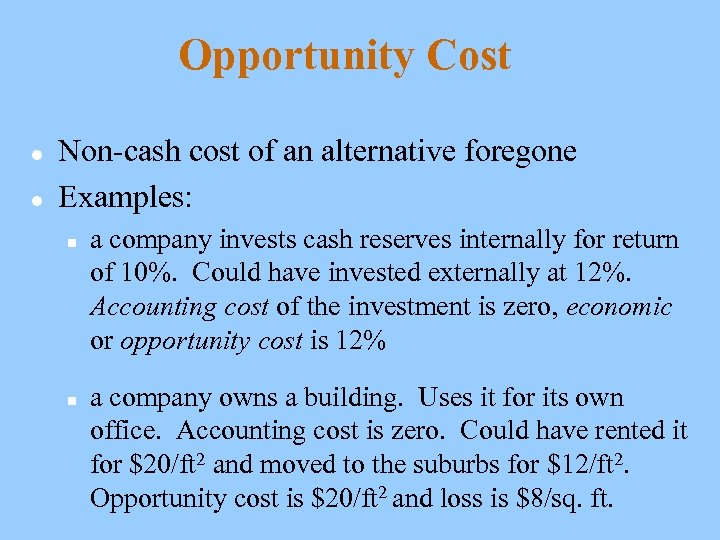Opportunity Cost l l Non-cash cost of an alternative foregone Examples: n n a company invests cash reserves internally for return of 10%. Could have invested externally at 12%. Accounting cost of the investment is zero, economic or opportunity cost is 12% a company owns a building. Uses it for its own office. Accounting cost is zero. Could have rented it for \$20/ft 2 and moved to the suburbs for \$12/ft 2. Opportunity cost is \$20/ft 2 and loss is \$8/sq. ft.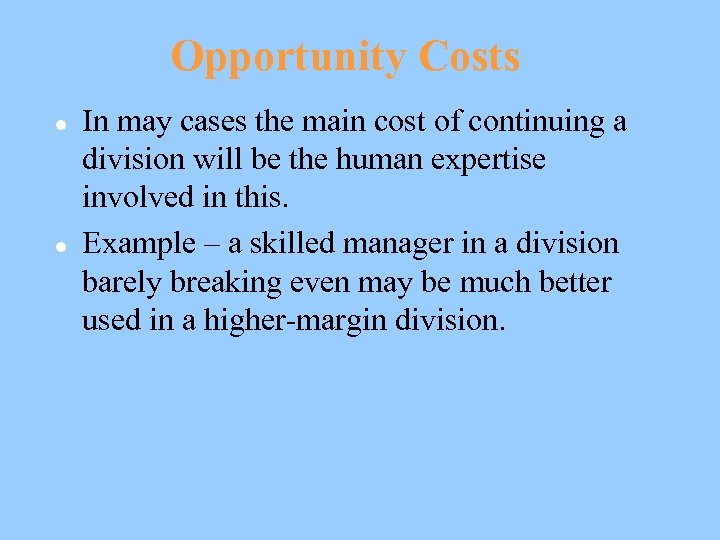Opportunity Costs l l In may cases the main cost of continuing a division will be the human expertise involved in this. Example – a skilled manager in a division barely breaking even may be much better used in a higher-margin division.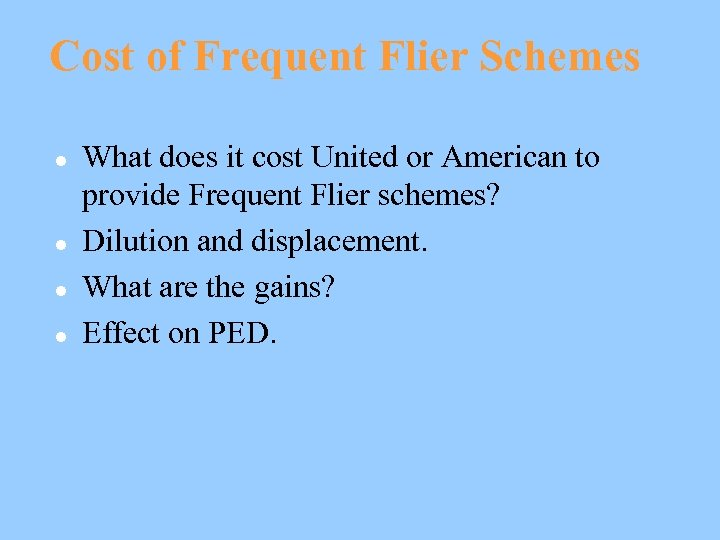Cost of Frequent Flier Schemes l l What does it cost United or American to provide Frequent Flier schemes? Dilution and displacement. What are the gains? Effect on PED.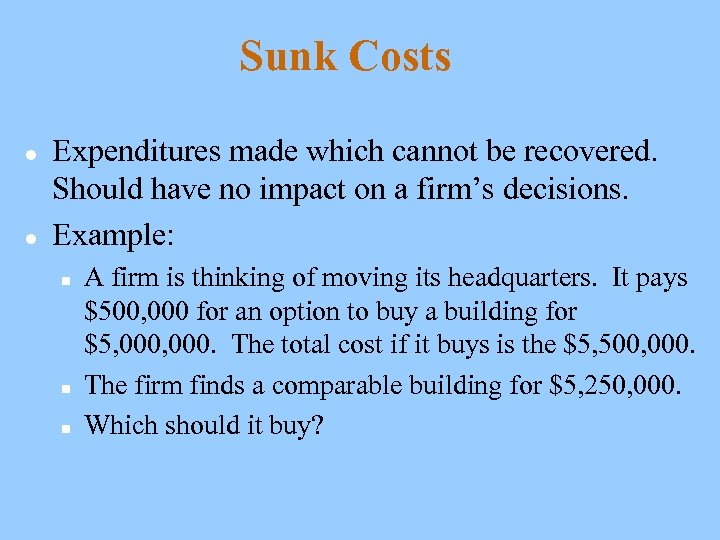Sunk Costs l l Expenditures made which cannot be recovered. Should have no impact on a firm’s decisions. Example: n n n A firm is thinking of moving its headquarters. It pays \$500, 000 for an option to buy a building for \$5, 000. The total cost if it buys is the \$5, 500, 000. The firm finds a comparable building for \$5, 250, 000. Which should it buy?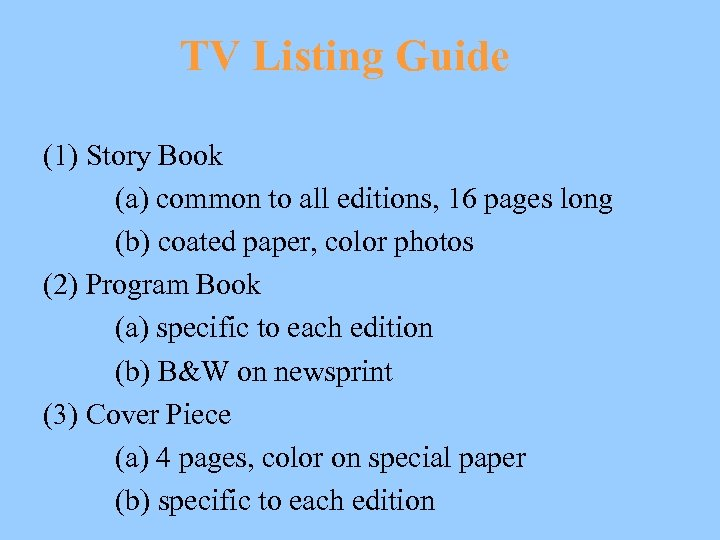TV Listing Guide (1) Story Book (a) common to all editions, 16 pages long (b) coated paper, color photos (2) Program Book (a) specific to each edition (b) B&W on newsprint (3) Cover Piece (a) 4 pages, color on special paper (b) specific to each edition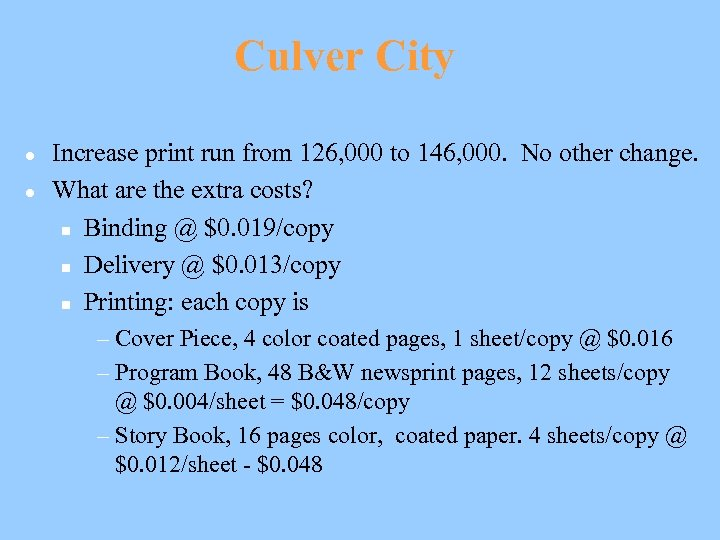Culver City l l Increase print run from 126, 000 to 146, 000. No other change. What are the extra costs? n Binding @ \$0. 019/copy n Delivery @ \$0. 013/copy n Printing: each copy is – Cover Piece, 4 color coated pages, 1 sheet/copy @ \$0. 016 – Program Book, 48 B&W newsprint pages, 12 sheets/copy @ \$0. 004/sheet = \$0. 048/copy – Story Book, 16 pages color, coated paper. 4 sheets/copy @ \$0. 012/sheet - \$0. 048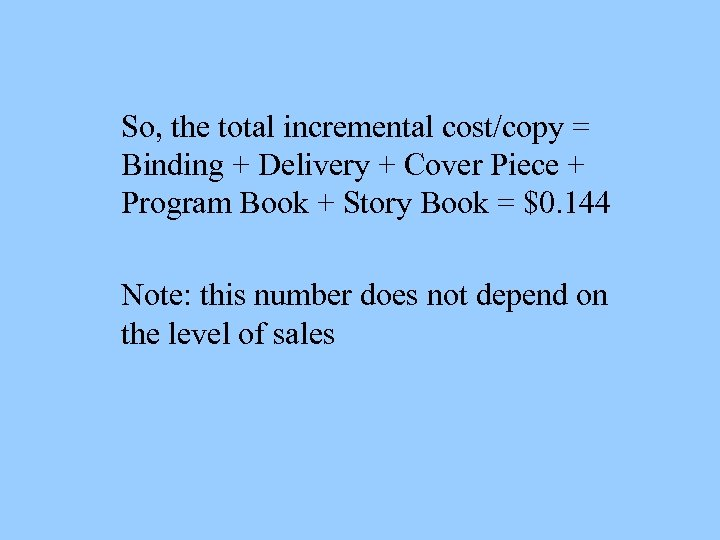So, the total incremental cost/copy = Binding + Delivery + Cover Piece + Program Book + Story Book = \$0. 144 Note: this number does not depend on the level of sales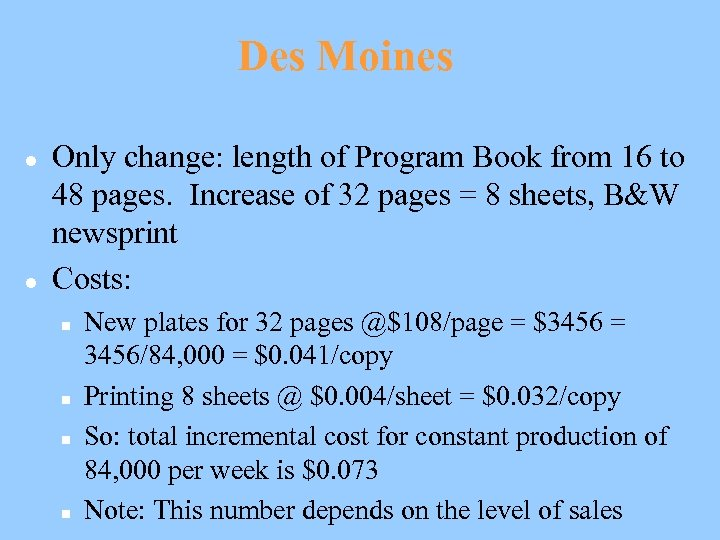Des Moines l l Only change: length of Program Book from 16 to 48 pages. Increase of 32 pages = 8 sheets, B&W newsprint Costs: n n New plates for 32 pages @\$108/page = \$3456 = 3456/84, 000 = \$0. 041/copy Printing 8 sheets @ \$0. 004/sheet = \$0. 032/copy So: total incremental cost for constant production of 84, 000 per week is \$0. 073 Note: This number depends on the level of sales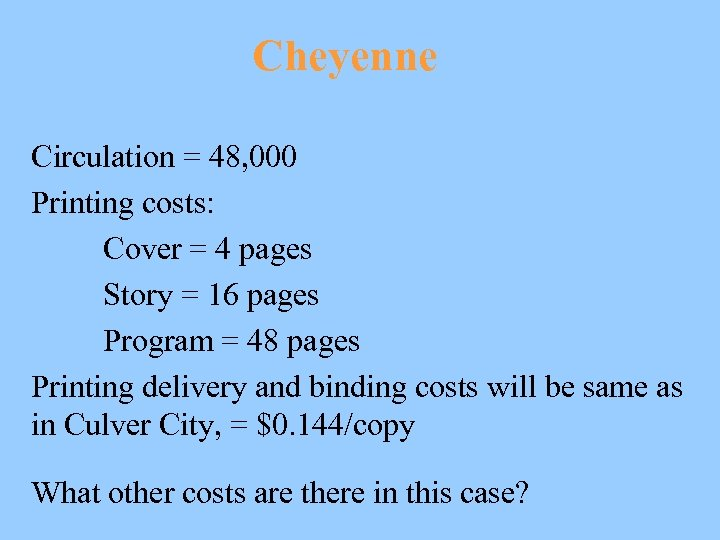Cheyenne Circulation = 48, 000 Printing costs: Cover = 4 pages Story = 16 pages Program = 48 pages Printing delivery and binding costs will be same as in Culver City, = \$0. 144/copy What other costs are there in this case?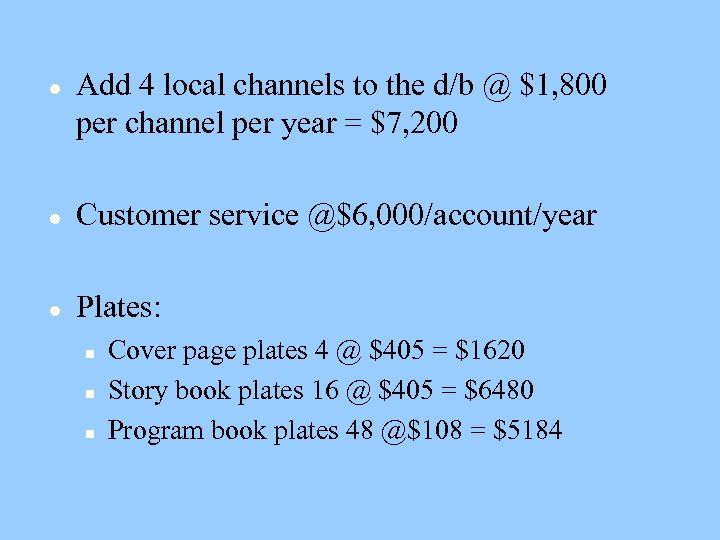l Add 4 local channels to the d/b @ \$1, 800 per channel per year = \$7, 200 l Customer service @\$6, 000/account/year l Plates: n n n Cover page plates 4 @ \$405 = \$1620 Story book plates 16 @ \$405 = \$6480 Program book plates 48 @\$108 = \$5184Makes total annual set up costs = \$13, 200 per year. To express this per copy divide by 52 x 48, 000 making \$0. 0053 a copy. Total weekly setup costs are \$13, 284. Per copy this is \$0. 276 Hence total incremental cost is \$0. 425 per copy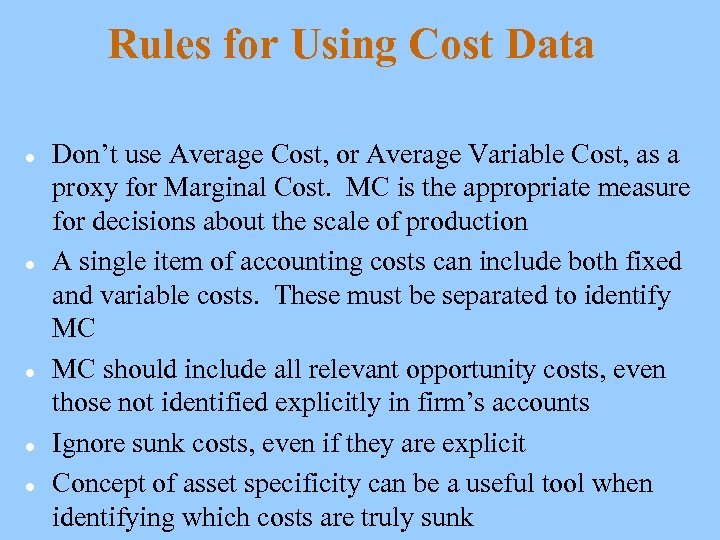Rules for Using Cost Data l l l Don’t use Average Cost, or Average Variable Cost, as a proxy for Marginal Cost. MC is the appropriate measure for decisions about the scale of production A single item of accounting costs can include both fixed and variable costs. These must be separated to identify MC MC should include all relevant opportunity costs, even those not identified explicitly in firm’s accounts Ignore sunk costs, even if they are explicit Concept of asset specificity can be a useful tool when identifying which costs are truly sunk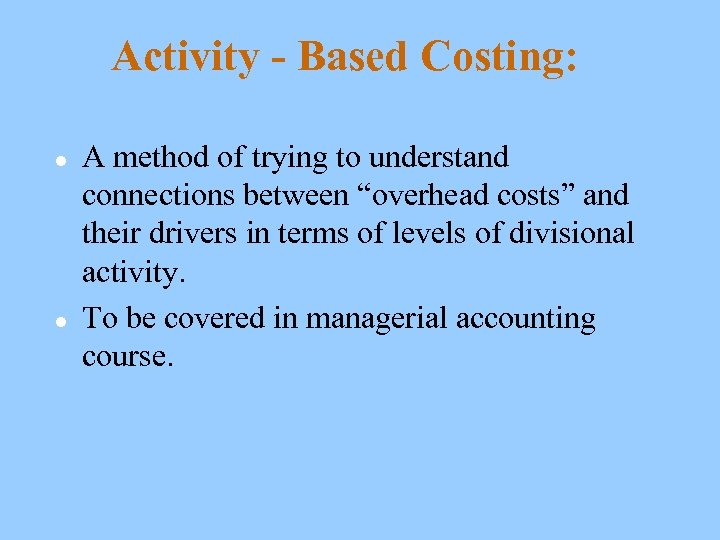Activity - Based Costing: l l A method of trying to understand connections between “overhead costs” and their drivers in terms of levels of divisional activity. To be covered in managerial accounting course.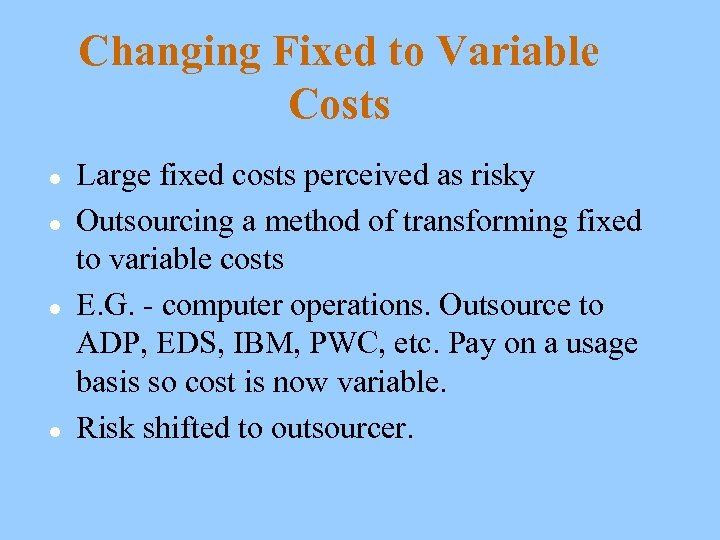Changing Fixed to Variable Costs l l Large fixed costs perceived as risky Outsourcing a method of transforming fixed to variable costs E. G. - computer operations. Outsource to ADP, EDS, IBM, PWC, etc. Pay on a usage basis so cost is now variable. Risk shifted to outsourcer.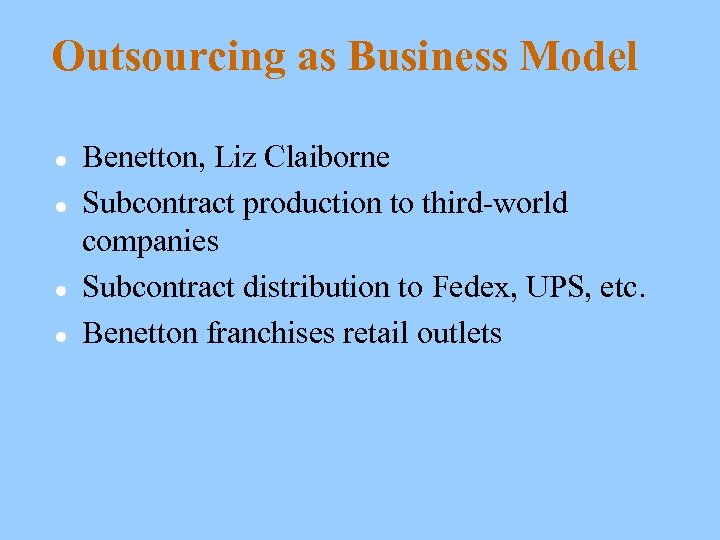Outsourcing as Business Model l l Benetton, Liz Claiborne Subcontract production to third-world companies Subcontract distribution to Fedex, UPS, etc. Benetton franchises retail outlets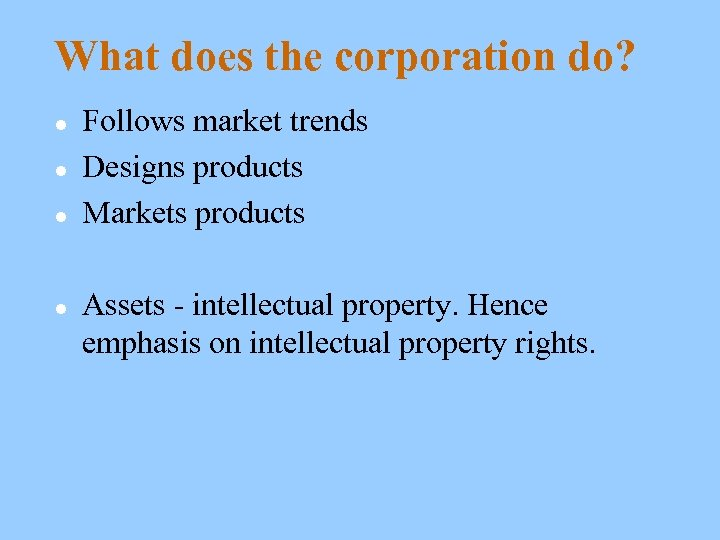What does the corporation do? l l Follows market trends Designs products Markets products Assets - intellectual property. Hence emphasis on intellectual property rights.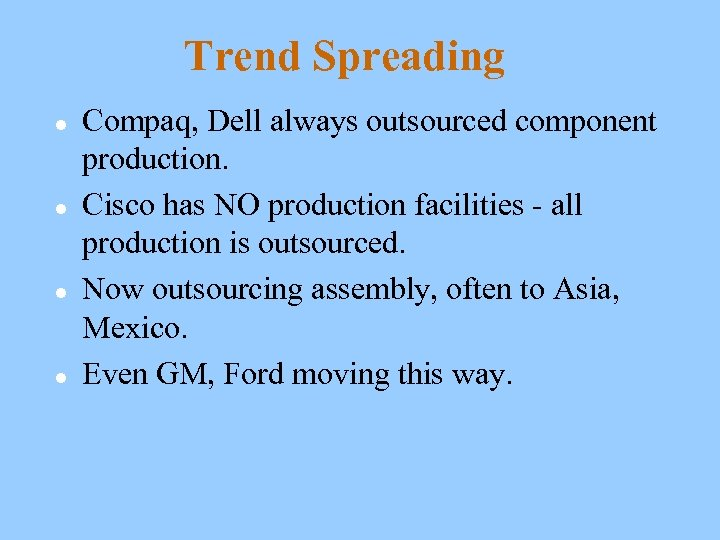Trend Spreading l l Compaq, Dell always outsourced component production. Cisco has NO production facilities - all production is outsourced. Now outsourcing assembly, often to Asia, Mexico. Even GM, Ford moving this way.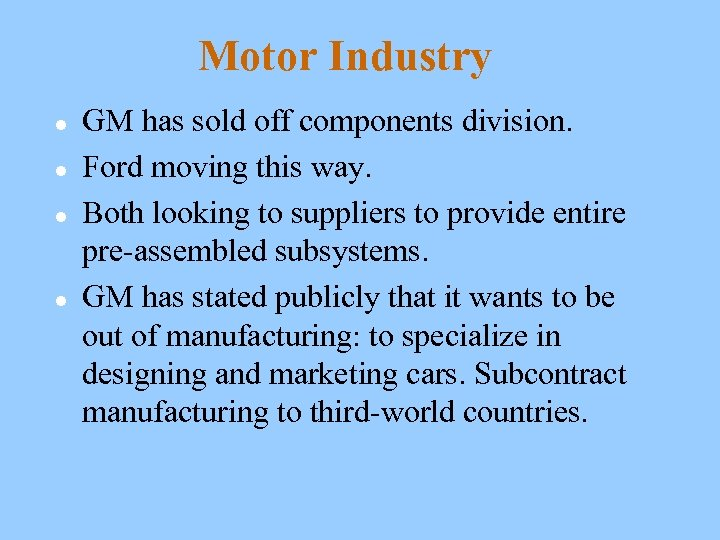Motor Industry l l GM has sold off components division. Ford moving this way. Both looking to suppliers to provide entire pre-assembled subsystems. GM has stated publicly that it wants to be out of manufacturing: to specialize in designing and marketing cars. Subcontract manufacturing to third-world countries.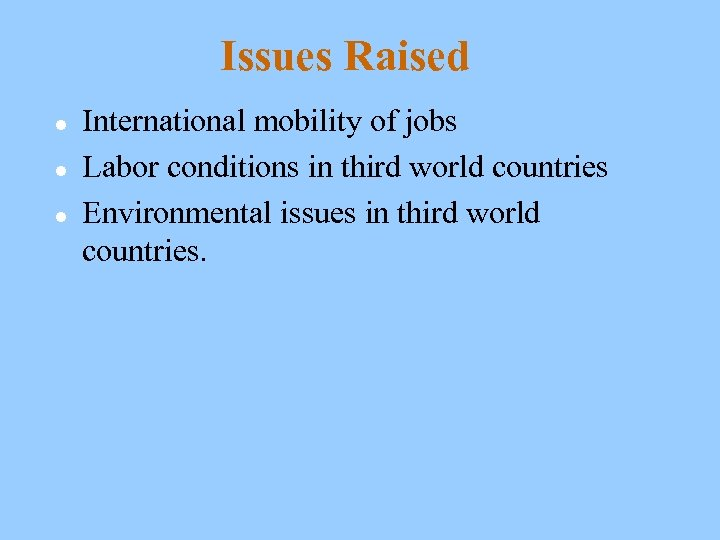Issues Raised l l l International mobility of jobs Labor conditions in third world countries Environmental issues in third world countries.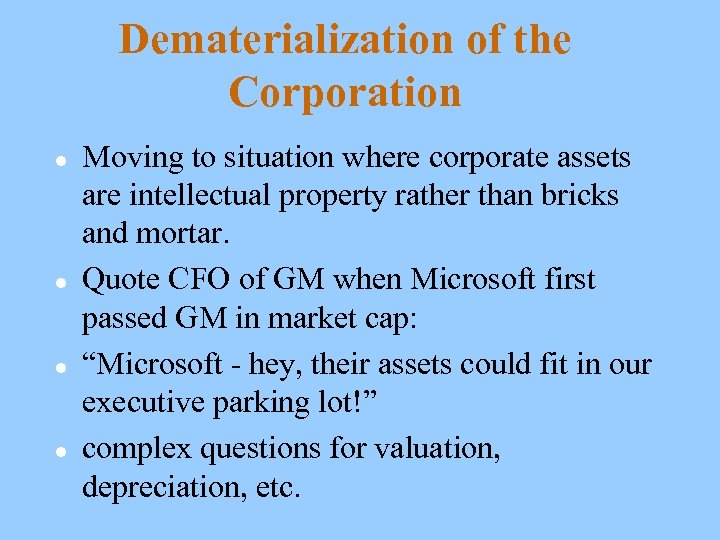Dematerialization of the Corporation l l Moving to situation where corporate assets are intellectual property rather than bricks and mortar. Quote CFO of GM when Microsoft first passed GM in market cap: “Microsoft - hey, their assets could fit in our executive parking lot!” complex questions for valuation, depreciation, etc.